# Random attractor for a stochastic viscous coupled Camassa-Holm equation

## Abstract

In this paper, we study the asymptotic dynamics of the stochastic viscous coupled Camassa-Holm equation with periodic boundary condition. We investigate the existence of a random attractor for the dynamical system associated with the equation. The random attractor is invariant and attracts every pull-back tempered random set under the forward flow. We establish the asymptotic compactness of the random dynamical system by compactness of embedding of Sobolev space.

## 1 Introduction

The Camassa-Holm equation

${u}_{t}-{u}_{xxt}+3u{u}_{x}-2{u}_{x}{u}_{xx}-u{u}_{xxx}=0$
(1.1)

models the unidirectional propagation of shallow water waves over a flat bottom . It has been paid considerable attention due to its rich nonlinear phenomenology. It is completely integrable  and it has stable solitons . It admits the peakons $u=c{e}^{|x-ct|}$ which is also stable [6, 7]. In [8, 9], it has been shown that (1.1) is locally well-posed for initial data ${u}_{0}\in {H}^{s}\left(\mathbb{R}\right)$ ($s>3/2$). More interestingly, there are a rich variety of global strong solutions and blow-up solutions . Xin and Zhang obtained the global existence of weak solution . Bressan and Constantin obtained the existence of global conservative and dissipative solutions [6, 12].

Some types of coupled Camassa-Holm equation appeared gradually and were investigated rapidly following (1.1). For example, Fu and Qu considered the following coupled Camassa-Holm equation :

$\begin{array}{r}{m}_{t}=2m{u}_{x}+{m}_{x}u+{\left(mv\right)}_{x}+n{v}_{x},\phantom{\rule{1em}{0ex}}m=u-{u}_{xx},\\ {n}_{t}=2n{v}_{x}+{n}_{x}v+{\left(nu\right)}_{x}+m{u}_{x},\phantom{\rule{1em}{0ex}}n=v-{v}_{xx},\end{array}$
(1.2)

which has peakon solitons in the form of a superposition of multipeakons. They investigated local well-posedness and blow-up solutions of (1.2) by means of Kato’s semigroup approach to nonlinear hyperbolic evolution equation and obtained a criterion and condition on the initial data guaranteeing the development of singularities in finite time for strong solutions of (1.2) by energy estimates. Moreover, an existence result for a class of local weak solutions was also given.

In , Tian and Xu investigated the existence of global attractor for a viscous Camassa-Holm equation as follows:

$\begin{array}{r}{m}_{t}-\epsilon {m}_{xx}=2m{u}_{x}+{m}_{x}u+{\left(mv\right)}_{x}+n{v}_{x},\phantom{\rule{1em}{0ex}}m=u-{u}_{xx},\\ {n}_{t}-\epsilon {n}_{xx}=2n{v}_{x}+{n}_{x}v+{\left(nu\right)}_{x}+m{u}_{x},\phantom{\rule{1em}{0ex}}n=v-{v}_{xx},\end{array}$
(1.3)

with the periodic boundary condition in ${H}^{2}$:

$\begin{array}{r}u\left(x,0\right)={u}_{0},\phantom{\rule{2em}{0ex}}v\left(x,0\right)={v}_{0},\\ u\left(0,t\right)=u\left(T,t\right),\phantom{\rule{2em}{0ex}}{u}_{x}\left(0,t\right)={u}_{x}\left(T,t\right),\phantom{\rule{2em}{0ex}}{u}_{xx}\left(0,t\right)={u}_{xx}\left(T,t\right),\\ v\left(0,t\right)=v\left(T,t\right),\phantom{\rule{2em}{0ex}}{v}_{x}\left(0,t\right)={v}_{x}\left(T,t\right),\phantom{\rule{2em}{0ex}}{v}_{xx}\left(0,t\right)={v}_{xx}\left(T,t\right).\end{array}$

The authors proved that (1.3) has a unique solution in infinite time interval by prior estimates and demonstrated its long time behavior which was described by a global attractor.

In , Tian, Xu and Zhou studied the existence of the global attractor for the viscous two-component Camassa-Holm equation:

$\begin{array}{r}{u}_{t}={u}_{xxt}-3u{u}_{x}+2{u}_{x}{u}_{xx}+u{u}_{xxx}+\epsilon \left(u-{u}_{xx}\right)-\rho {\rho }_{x},\\ {\rho }_{t}=-{\left(u\rho \right)}_{x}+\epsilon {\rho }_{xx},\end{array}$
(1.4)

with the periodic boundary condition in ${H}^{2}\left(\left[0,L\right]\right)$:

$\begin{array}{r}u\left(x,0\right)={u}_{0}\left(x\right),\phantom{\rule{2em}{0ex}}\rho \left(x,0\right)={\rho }_{0},\\ u\left(0,t\right)=u\left(L,t\right),\phantom{\rule{2em}{0ex}}{u}_{x}\left(0,t\right)={u}_{x}\left(L,t\right),\phantom{\rule{2em}{0ex}}{u}_{xx}\left(0,t\right)={u}_{xx}\left(L,t\right),\\ \rho \left(0,t\right)=\rho \left(L,t\right),\phantom{\rule{2em}{0ex}}{\rho }_{x}\left(0,t\right)={\rho }_{x}\left(L,t\right),\phantom{\rule{2em}{0ex}}{\rho }_{xx}\left(0,t\right)={\rho }_{xx}\left(L,t\right).\end{array}$

They considered the well-posedness and discussed the existence of global solution of (1.4) in ${H}^{2}\left(\left[0,L\right]\right)×{H}^{2}\left(\left[0,L\right]\right)$ by using prior estimates. Finally, they showed the long time behavior of solution and obtained the existence of global attractor of (1.4).

Motivated by the persistence of the global attractor under a white noise, in our paper, we will investigate the long time behavior and the existence of the global random attractor of stochastic viscous coupled Camassa-Holm equation:

$\begin{array}{r}{m}_{t}-\epsilon {m}_{xx}=2m{u}_{x}+{m}_{x}u+{\left(mv\right)}_{x}+n{v}_{x}+h{W}_{t},\phantom{\rule{1em}{0ex}}m=u-{u}_{xx},\\ {n}_{t}-\epsilon {n}_{xx}=2n{v}_{x}+{n}_{x}v+{\left(nu\right)}_{x}+m{u}_{x},\phantom{\rule{1em}{0ex}}n=v-{v}_{xx},\end{array}$
(1.5)

with initial and periodic boundary condition

$\begin{array}{r}u\left(x,0\right)={u}_{0},\phantom{\rule{2em}{0ex}}v\left(x,0\right)={v}_{0},\\ u\left(0,t\right)=u\left(T,t\right),\phantom{\rule{2em}{0ex}}{u}_{x}\left(0,t\right)={u}_{x}\left(T,t\right),\phantom{\rule{2em}{0ex}}{u}_{xx}\left(0,t\right)={u}_{xx}\left(T,t\right),\\ v\left(0,t\right)=v\left(T,t\right),\phantom{\rule{2em}{0ex}}{v}_{x}\left(0,t\right)={v}_{x}\left(T,t\right),\phantom{\rule{2em}{0ex}}{v}_{xx}\left(0,t\right)={v}_{xx}\left(T,t\right),\end{array}$

where $t>0$, $x\in \left[0,T\right]$, $T>0$, ${u}_{0},{v}_{0}\in {L}^{2}\left(\left[0,T\right]\right)$. $h=h\left(x\right)$ is a given function defined on $\left[0,T\right]$, which described in the following. $W\left(t\right)$ is a two-side real-valued Wiener process on a probability space which will be specified later.

A system is usually uncertain in reality due to some external noise, which is random. The random effects are considered not only as compensations for the defects in some deterministic models, but also rather essential phenomena .

Attractor is an important concept to describe long time behavior of solutions for a given system. It is known that the long time behavior of random systems is captured by a pullback random attractor, which was introduced in [17, 21, 22] as an extension of attractor theory of deterministic system in . The existence of random attractors for stochastic differential equations has been studied extensively by many authors . To our best knowledge, the problem of random attractor for (1.5) has not been discussed. We think it is a significant work to obtain a random attractor for the system.

The paper is organized as follows. In the next section, we review the pullback random attractor theory for random dynamical systems and some lemmas. In Section 3, we define a random dynamical system for the stochastic viscous coupled Camassa-Holm equation. Then we derive the uniform estimates of solutions in Section 4. These estimates are necessary for proving the existence of bounded random absorbing sets and the asymptotic compactness of the random dynamical system and prove the existence of a pullback random attractor in ${H}^{2}\left(\left[0,T\right]\right)×{H}^{2}\left(\left[0,T\right]\right)$. We conclude that the global attractor persists under a white noise.

## 2 Preliminaries

In this section, we recall some basic concepts related to random attractors for stochastic dynamical systems and some basic inequalities, which refer to [1618, 21, 36] for more details.

Let $\left(X,{\parallel \cdot \parallel }_{X}\right)$ be a separable Hilbert space with Borel σ-algebra $\mathcal{B}\left(X\right)$, and $\left(\mathrm{\Omega },\mathcal{F},P\right)$ be a probability space.

Definition 2.1 $\left(\mathrm{\Omega },\mathcal{F},P,{\left({\theta }_{t}\right)}_{t\in \mathbb{R}}\right)$ is called a metric dynamical system if $\theta :\mathbb{R}×\mathrm{\Omega }\to \mathrm{\Omega }$ is $\left(\mathcal{B}\left(X\right)×\mathcal{F},\mathcal{F}\right)$-measurable, ${\theta }_{0}$ is the identity on Ω, ${\theta }_{s+t}={\theta }_{t}\circ {\theta }_{s}$ for all $s,t\in \mathbb{R}$ and ${\theta }_{t}P=P$ for all $t\in \mathbb{R}$.

Definition 2.2 A continuous random dynamical system (RDS) on X over a metric dynamical system $\left(\mathrm{\Omega },\mathcal{F},P,{\left({\theta }_{t}\right)}_{t\in \mathbb{R}}\right)$ is a mapping

$\mathrm{\Phi }:{\mathbb{R}}^{+}×\mathrm{\Omega }×X\to X,\phantom{\rule{2em}{0ex}}\left(t,\omega ,x\right)↦\mathrm{\Phi }\left(t,\omega ,x\right),$

which is $\left(\mathcal{B}\left({\mathbb{R}}^{+}\right)×\mathcal{F}×\mathcal{B}\left(X\right),\mathcal{B}\left(X\right)\right)$-measurable and satisfies, for P-a.e. $\omega \in \mathrm{\Omega }$,

1. (i)

$\mathrm{\Phi }\left(0,\omega ,\cdot \right)$ is the identity on X;

2. (ii)

$\mathrm{\Phi }\left(t+s,\omega ,\cdot \right)=\mathrm{\Phi }\left(t,{\theta }_{s}\omega ,\cdot \right)\circ \mathrm{\Phi }\left(s,\omega ,\cdot \right)$ for all $t,s\in {\mathbb{R}}^{+}$;

3. (iii)

$\mathrm{\Phi }\left(t,\omega ,\cdot \right):X\to X$ is continuous for all $t\in {\mathbb{R}}^{+}$.

Hereafter, we assume that Φ is a continuous RDS on X over $\left(\mathrm{\Omega },\mathcal{F},P,{\left({\theta }_{t}\right)}_{t\in \mathbb{R}}\right)$.

Definition 2.3 A random bounded set ${\left\{B\left(\omega \right)\right\}}_{\omega \in \mathrm{\Omega }}$ of X is called tempered with respect to ${\left({\theta }_{t}\right)}_{t\in \mathbb{R}}$ if for P-a.e. $\omega \in \mathrm{\Omega }$,

$\underset{t\to +\mathrm{\infty }}{lim}{e}^{-\beta t}d\left(B\left({\theta }_{-t}\omega \right)\right)=0,\phantom{\rule{1em}{0ex}}\mathrm{\forall }\beta >0,$

where $d\left(B\right)={sup}_{x\in B}{\parallel x\parallel }_{X}$.

Definition 2.4 Let $\mathcal{D}$ be a collection of random subsets of X. Then $\mathcal{D}$ is called inclusion-closed if $D={\left\{D\left(\omega \right)\right\}}_{\omega \in \mathrm{\Omega }}\in \mathcal{D}$ and $\stackrel{˜}{D}=\left\{\stackrel{˜}{D}\left(\omega \right)\subseteq X:\omega \in \mathrm{\Omega }\right\}$ with $\stackrel{˜}{D}\subseteq D\left(\omega \right)$ for all $\omega \in \mathrm{\Omega }$ imply that $\stackrel{˜}{D}\in \mathcal{D}$.

Definition 2.5 A random set ${\left\{K\left(\omega \right)\right\}}_{\omega \in \mathrm{\Omega }}\in \mathcal{D}$ is called an absorbing set of Φ in $\mathcal{D}$ if for every $B\in \mathcal{D}$ and P-a.e. $\omega \in \mathrm{\Omega }$, there exists ${t}_{B}\left(\omega \right)>0$ such that

$\mathrm{\Phi }\left(t,{\theta }_{-t}\omega ,B\left({\theta }_{-t}\omega \right)\right)\in K\left(\omega \right),\phantom{\rule{1em}{0ex}}\mathrm{\forall }t\ge {t}_{B}\left(\omega \right).$

Definition 2.6 The RDS Φ is said to be $\mathcal{D}$-pullback asymptotically compact in X if for P-a.e. $\omega \in \mathrm{\Omega }$, ${\left\{\mathrm{\Phi }\left({t}_{n},{\theta }_{-{t}_{n}}\omega ,{x}_{n}\right)\right\}}_{n=1}^{\mathrm{\infty }}$ has a convergent subsequence in X whenever ${t}_{n}\to \mathrm{\infty }$, and ${x}_{n}\in B\left({\theta }_{-{t}_{n}}\omega \right)$ with ${\left\{B\left(\omega \right)\right\}}_{\omega \in \mathrm{\Omega }}\in \mathcal{D}$.

Definition 2.7 A random set ${\left\{\mathcal{A}\left(\omega \right)\right\}}_{\omega \in \mathrm{\Omega }}$ is called a $\mathcal{D}$-pullback attractor (or $\mathcal{D}$-random attractor) for Ω if the following conditions are satisfied, for P-a.e. $\omega \in \mathrm{\Omega }$,

1. (i)

${\left\{\mathcal{A}\left(\omega \right)\right\}}_{\omega \in \mathrm{\Omega }}$ is compact, and $\omega ↦d\left(x,\mathcal{A}\left(\omega \right)\right)$ is measurable for every $x\in X$;

2. (ii)

${\left\{\mathcal{A}\left(\omega \right)\right\}}_{\omega \in \mathrm{\Omega }}$ is invariant, that is,

$\mathrm{\Phi }\left(t,\omega ,\mathcal{A}\left(\omega \right)\right)=\mathcal{A}\left({\theta }_{t}\omega \right),\phantom{\rule{1em}{0ex}}\mathrm{\forall }t\ge 0;$
3. (iii)

${\left\{\mathcal{A}\left(\omega \right)\right\}}_{\omega \in \mathrm{\Omega }}$ attracts every set in $\mathcal{D}$, that is, for every $B={\left\{B\left(\omega \right)\right\}}_{\omega \in \mathrm{\Omega }}\in \mathcal{D}$,

$\underset{t\to \mathrm{\infty }}{lim}d\left(\mathrm{\Phi }\left(t,{\theta }_{-t}\omega ,B\left({\theta }_{-t}\omega \right)\right),\mathcal{A}\left(\omega \right)\right)=0,$

where d is the Hausdorff semi-metric given by $d\left(Y,Z\right)={sup}_{y\in Y}{inf}_{z\in Z}{\parallel y-z\parallel }_{X}$ for any $Y\subseteq X$ and $Z\subseteq X$.

The following existence result on a random attractor for a continuous RDS can be found in [18, 28].

Theorem 2.1 Let $\mathcal{D}$ be an inclusion-closed collection of random subsets of X and Φ a continuous RDS on X over $\left(\mathrm{\Omega },\mathcal{F},P,{\left({\theta }_{t}\right)}_{t\in \mathbb{R}}\right)$. Suppose that ${\left\{K\left(\omega \right)\right\}}_{\omega \in \mathrm{\Omega }}$ is closed absorbing set of Φ in $\mathcal{D}$ and Φ is $\mathcal{D}$-pullback asymptotically compact in X. Then Φ has a unique $\mathcal{D}$-pullback attractor ${\left\{\mathcal{A}\left(\omega \right)\right\}}_{\omega \in \mathrm{\Omega }}$ which is given by

$\mathcal{A}\left(\omega \right)=\bigcap _{\tau \ge 0}\overline{\bigcup _{t\ge \tau }\mathrm{\Phi }\left(t,{\theta }_{-t}\omega ,K\left({\theta }_{-t}\omega \right)\right)}.$

Some basic inequalities which will be used frequently in the following consideration are presented as follows.

Lemma 2.1 (Gagliardo-Nirenberg inequality)

Suppose that $u\in {L}^{q}\left(\mathrm{\Sigma }\right)\cap {W}_{0}^{m,r}\left(\mathrm{\Sigma }\right)$, $\mathrm{\Sigma }\subset {\mathbb{R}}^{k}$, $1\le q,r\le \mathrm{\infty }$, $0\le j\le m$, $\frac{j}{m}\le \alpha <1$, $1\le p<\mathrm{\infty }$, and there exists a constant c, such that

${\parallel {D}^{j}u\parallel }_{{L}^{p}}\le c{\parallel {D}^{m}u\parallel }_{{L}^{r}}^{\alpha }{\parallel u\parallel }_{{L}^{q}}^{1-\alpha },\phantom{\rule{2em}{0ex}}\frac{1}{p}=\frac{j}{k}+\alpha \left(\frac{1}{r}-\frac{m}{k}\right)+\left(1-\alpha \right)\frac{1}{q}.$

Lemma 2.2 (Poincaré inequality)

Assume that $1\le p\le \mathrm{\infty }$ and that Σ is a bounded connected open subset of the n-dimensional Euclidean space ${\mathbb{R}}^{n}$ with a Lipschitz boundary (i.e., Σ is a Lipschitz domain). Then there exists a constant ${c}_{0}$, depending only on Σ and p, such that for every function u in the Sobolev space ${W}^{1,p}\left(\mathrm{\Sigma }\right)$,

${\parallel u-{u}_{\mathrm{\Sigma }}\parallel }_{{L}^{p}\left(\mathrm{\Sigma }\right)}\le {c}_{0}{\parallel \mathrm{\nabla }u\parallel }_{{L}^{p}\left(\mathrm{\Sigma }\right)},$

where ${u}_{\mathrm{\Sigma }}=\frac{1}{|\mathrm{\Sigma }|}{\int }_{\mathrm{\Sigma }}u\left(y\right)\phantom{\rule{0.2em}{0ex}}dy$.

Lemma 2.3 (Young inequality)

$ab\le \left(\delta {a}^{p}/p\right)+\left({\delta }^{-q/p}{b}^{q}/q\right)\le \delta {a}^{p}+{\delta }^{-q/p}{b}^{q}$, where $1, $\left(1/p\right)+\left(1/q\right)=1$. As $p=q=2$, one has $ab\le \left(\delta {a}^{2}/2\right)+\left({b}^{2}/2\delta \right)\le \left(\delta {a}^{2}\right)+\left({b}^{2}/\delta \right)$.

## 3 Stochastic viscous coupled Camassa-Holm equation

For convenience, we introduce some marks. We denote ${\parallel \cdot \parallel }_{p}$ and ${\parallel \cdot \parallel }_{\mathrm{\infty }}$ the norms in ${L}^{p}\left(\left[0,T\right]\right)$ and ${L}^{\mathrm{\infty }}\left(\left[0,T\right]\right)$ respectively. We denote $H=\left\{u\in {L}^{2}\left(\left[0,T\right]\right)|u\left(0,t\right)=u\left(T,t\right),{u}_{x}\left(0,t\right)={u}_{x}\left(T,t\right),{u}_{xx}\left(0,t\right)={u}_{xx}\left(T,t\right)\right\}$ and $V=\left\{u\in {H}^{1}\left(\left[0,T\right]\right)|u\left(0,t\right)=u\left(T,t\right),{u}_{x}\left(0,t\right)={u}_{x}\left(T,t\right),{u}_{xx}\left(0,t\right)={u}_{xx}\left(T,t\right)\right\}$. The Laplace operator Δ is an isomorphism from $V\cap {H}^{2}\left(\left[0,T\right]\right)$ to H. The eigenvalues of Δ has the form $\left({k}_{1}^{2}+{k}_{2}^{2}\right)\left(2\pi /T\right)$ where ${k}_{1},{k}_{2}\in N$ and ${k}_{1}^{2}+{k}_{2}^{2}\ne 0$. Then the Poincaré inequality is simplified down to $\lambda {\parallel u\parallel }_{2}^{2}\le {\parallel \mathrm{\nabla }u\parallel }_{2}^{2}$, where $u\in V$ and λ denotes the first eigenvalue of Δ.

In this section, we discuss the existence of a continuous random dynamical system for the stochastic viscous coupled Camassa-Holm equation defined on $\left[0,T\right]$. Consider the following stochastic equation:(3.1)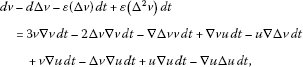(3.2)

with initial and periodic boundary condition(3.3)(3.4)(3.5)

where $h\left(x\right)\in V\cap {H}^{2}\left(\left[0,T\right]\right)$. W is a two-sided real-valued Wiener process on a probability space, which will be determined below.

In the sequel, we consider the probability space $\left(\mathrm{\Omega },\mathcal{F},P\right)$ where

$\mathrm{\Omega }=\left\{\omega \in C\left(\mathbb{R},\mathbb{R}\right):\omega \left(0\right)=0\right\},$

is the Borel σ-algebra induced by the compact-open topology of Ω, and P the corresponding Wiener measure on $\left(\mathrm{\Omega },\mathcal{F}\right)$. Define the time shift by

${\theta }_{t}\omega \left(\cdot \right)=\omega \left(\cdot +t\right)-\omega \left(t\right),\phantom{\rule{1em}{0ex}}\omega \in \mathrm{\Omega },t\in \mathbb{R}.$

Then $\left(\mathrm{\Omega },\mathcal{F},P,{\left({\theta }_{t}\right)}_{t\in \mathbb{R}}\right)$ is a metric dynamical system. We need to convert the stochastic equation (3.1) with a random term into a deterministic one with a random parameter. Then we consider the stationary solutions of the one-dimensional equation:

$dy+\alpha y\phantom{\rule{0.2em}{0ex}}dt=dW\left(t\right).$
(3.6)

The solution to (3.6) is given by

$y\left({\theta }_{t}\omega \right)=-\alpha {\int }_{-\mathrm{\infty }}^{0}{e}^{\alpha \tau }\left({\theta }_{\tau }\omega \right)\phantom{\rule{0.2em}{0ex}}d\tau ,\phantom{\rule{1em}{0ex}}t\in \mathbb{R}.$
(3.7)

It’s known that there exists a ${\theta }_{t}$-invariant set $\stackrel{˜}{\mathrm{\Omega }}\subseteq \mathrm{\Omega }$ of full P measure such that $y\left({\theta }_{t}\omega \right)$ is continuous in t for every $\omega \in \stackrel{˜}{\mathrm{\Omega }}$, and the random variable $|y\left(\omega \right)|$ is tempered [16, 17, 21, 28]. We give some properties of the process as follows.

Lemma 3.1 For the Ornstein-Uhlenbeck process $y\left({\theta }_{t}\omega \right)$, we have the following results(3.8)(3.9)(3.10)(3.11)

Define $z\left({\theta }_{t}\omega \right)={\left(I-\mathrm{\Delta }\right)}^{-1}hy\left({\theta }_{t}\omega \right)$, where the domain of the Laplace operator Δ is $V\cap {H}^{2}\left(\left[0,T\right]\right)$. By (3.6) we find that

$dz-d\left(\mathrm{\Delta }z\right)+\alpha \left(z-\mathrm{\Delta }z\right)\phantom{\rule{0.2em}{0ex}}dt=h\phantom{\rule{0.2em}{0ex}}dW.$
(3.12)

Substituting $u\left(t,\omega \right)$ in (3.1)-(3.2) for $u\left(t,\omega \right)+z\left({\theta }_{t}\omega \right)$, we have that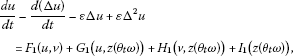(3.13)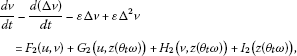(3.14)

where

$\left\{\begin{array}{c}{F}_{1}\left(u,v\right)=3u\mathrm{\nabla }u-2\mathrm{\Delta }u\mathrm{\nabla }u-u\mathrm{\nabla }\mathrm{\Delta }u+\mathrm{\nabla }uv-\mathrm{\nabla }\mathrm{\Delta }uv\hfill \\ \phantom{{F}_{1}\left(u,v\right)=}+u\mathrm{\nabla }v-\mathrm{\Delta }u\mathrm{\nabla }v+v\mathrm{\nabla }v-\mathrm{\nabla }v\mathrm{\Delta }v,\hfill \\ {G}_{1}\left(u,z\left({\theta }_{t}\omega \right)\right)=3u\mathrm{\nabla }z\left({\theta }_{t}\omega \right)+3\mathrm{\nabla }uz\left({\theta }_{t}\omega \right)-2\mathrm{\Delta }u\mathrm{\nabla }z\left({\theta }_{t}\omega \right)-2\mathrm{\nabla }u\mathrm{\Delta }z\left({\theta }_{t}\omega \right)\hfill \\ \phantom{{G}_{1}\left(u,z\left({\theta }_{t}\omega \right)\right)=}-u\mathrm{\nabla }\mathrm{\Delta }z\left({\theta }_{t}\omega \right)-\mathrm{\nabla }\mathrm{\Delta }uz\left({\theta }_{t}\omega \right),\hfill \\ {H}_{1}\left(v,z\left({\theta }_{t}\omega \right)\right)=v\mathrm{\nabla }z\left({\theta }_{t}\omega \right)-v\mathrm{\nabla }\mathrm{\Delta }z\left({\theta }_{t}\omega \right)+\mathrm{\nabla }vz\left({\theta }_{t}\omega \right)-\mathrm{\nabla }v\mathrm{\Delta }z\left({\theta }_{t}\omega \right),\hfill \\ {I}_{1}\left(z\left({\theta }_{t}\omega \right)\right)=3z\left({\theta }_{t}\omega \right)\mathrm{\nabla }z\left({\theta }_{t}\omega \right)+\epsilon \mathrm{\Delta }z\left({\theta }_{t}\omega \right)-\epsilon {\mathrm{\Delta }}^{2}z\left({\theta }_{t}\omega \right)-2\mathrm{\Delta }z\left({\theta }_{t}\omega \right)\mathrm{\nabla }z\left({\theta }_{t}\omega \right)\hfill \\ \phantom{{I}_{1}\left(z\left({\theta }_{t}\omega \right)\right)=}-z\left({\theta }_{t}\omega \right)\mathrm{\nabla }\mathrm{\Delta }z\left({\theta }_{t}\omega \right)+\alpha z\left({\theta }_{t}\omega \right)-\alpha \mathrm{\Delta }z\left({\theta }_{t}\omega \right),\hfill \\ {F}_{2}\left(u,v\right)=3v\mathrm{\nabla }v-2\mathrm{\Delta }v\mathrm{\nabla }v-v\mathrm{\nabla }\mathrm{\Delta }v+u\mathrm{\nabla }v-u\mathrm{\nabla }\mathrm{\Delta }v\hfill \\ \phantom{{F}_{2}\left(u,v\right)=}+v\mathrm{\nabla }u-\mathrm{\Delta }v\mathrm{\nabla }u+u\mathrm{\nabla }u-\mathrm{\nabla }u\mathrm{\Delta }u,\hfill \\ {G}_{2}\left(u,z\left({\theta }_{t}\omega \right)\right)=u\mathrm{\nabla }z\left({\theta }_{t}\omega \right)+\mathrm{\nabla }uz\left({\theta }_{t}\omega \right)-\mathrm{\Delta }u\mathrm{\nabla }z\left({\theta }_{t}\omega \right)-\mathrm{\nabla }u\mathrm{\Delta }z\left({\theta }_{t}\omega \right),\hfill \\ {H}_{2}\left(v,z\left({\theta }_{t}\omega \right)\right)=\mathrm{\nabla }vz\left({\theta }_{t}\omega \right)-\mathrm{\nabla }\mathrm{\Delta }vz\left({\theta }_{t}\omega \right)+v\mathrm{\nabla }z\left({\theta }_{t}\omega \right)-\mathrm{\Delta }v\mathrm{\nabla }z\left({\theta }_{t}\omega \right),\hfill \\ {I}_{2}\left(z\left({\theta }_{t}\omega \right)\right)=z\left({\theta }_{t}\omega \right)\mathrm{\nabla }z\left({\theta }_{t}\omega \right)-\mathrm{\Delta }z\left({\theta }_{t}\omega \right)\mathrm{\nabla }z\left({\theta }_{t}\omega \right),\hfill \end{array}$

with the boundary condition(3.15)(3.16)

and initial condition

$u\left(x,0,\omega \right)={u}_{0}\left(x,\omega \right),\phantom{\rule{2em}{0ex}}v\left(x,0,\omega \right)={v}_{0}\left(x,\omega \right).$
(3.17)

We will consider (3.13)-(3.17) for $\omega \in \stackrel{˜}{\mathrm{\Omega }}$ and write $\stackrel{˜}{\mathrm{\Omega }}$ as Ω from now on.

Let $E={H}^{2}\left(\left[0,T\right]\right)×{H}^{2}\left(\left[0,T\right]\right)$, by a Galerkin method as in [14, 15], it can be proved that for P-a.e. $\omega \in \mathrm{\Omega }$ and for the initial condition ${\left({u}_{0},{v}_{0}\right)}^{T}\in E$, problem (3.13)-(3.17) has a unique global solution ${\left(u\left(\cdot ,\omega \right),v\left(\cdot ,\omega \right)\right)}^{T}\in C\left(\left[0,+\mathrm{\infty }\right),E\right)$ with ${\left(u\left(0,\omega \right),v\left(0,\omega \right)\right)}^{T}={\left({u}_{0},{v}_{0}\right)}^{T}$. Further, the solution ${\left(u\left(t,\omega ,{u}_{0}\right),v\left(t,\omega ,{v}_{0}\right)\right)}^{T}$ is continuous with respect to ${\left({u}_{0},{v}_{0}\right)}^{T}$ in E for all $t\ge 0$. Hence, the solution mapping $\mathrm{\Phi }:{\mathbb{R}}^{+}×\mathrm{\Omega }×E\to E$ defined by

$\mathrm{\Phi }\left(t,\omega ,{\varphi }_{0}\right)={\left(u\left(t,\omega ,{u}_{0}\right),v\left(t,\omega ,{v}_{0}\right)\right)}^{T},\phantom{\rule{1em}{0ex}}\mathrm{\forall }\left(t,\omega ,{\varphi }_{0}\right)\in {\mathbb{R}}^{+}×\mathrm{\Omega }×E$
(3.18)

generates a continuous random dynamical system, where ${\varphi }_{0}={\left({u}_{0},{v}_{0}\right)}^{T}$.

Now we introduce a homeomorphism $P\left({\theta }_{t}\omega \right){\left(u,v\right)}^{T}={\left(u+z\left({\theta }_{t}\omega \right),v\right)}^{T}$, ${\left(u,v\right)}^{T}\in E$ whose inverse homeomorphism ${P}^{-1}\left({\theta }_{t}\omega \right){\left(u,v\right)}^{T}={\left(u-z\left({\theta }_{t}\omega \right),v\right)}^{T}$. Then the transformation

$\mathrm{\Psi }\left(t,\omega \right)=P\left({\theta }_{t}\omega \right)\mathrm{\Phi }\left(t,\omega \right){P}^{-1}\left({\theta }_{t}\omega \right)$
(3.19)

generates a random dynamical system associated with (3.1)-(3.5). Note that the two random dynamical systems are equivalent by (3.19). It is easy to check that $\mathrm{\Psi }\left(t,\omega \right)$ has a random attractor provided $\mathrm{\Phi }\left(t,\omega \right)$ possesses a random attractor. So, we only need to consider the random dynamical system $\mathrm{\Phi }\left(t,\omega \right)$.

## 4 Uniform estimates of solution

In this section, we derive uniform estimates on the solution of the stochastic viscous coupled Camassa-Hlom equation when $t\to +\mathrm{\infty }$. These estimates are necessary for proving the existence of bounded absorbing sets and the asymptotic compactness of the random dynamical system. From now on, we always assume that $\mathcal{D}$ is the collection of tempered random subsets of E.

As a beginning, we estimate the process $z\left({\theta }_{t}\omega \right)$. By employing Gagliardo-Nirenberg’s inequality and Cauchy-Schwatz’s inequality, we have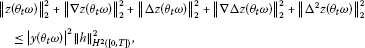(4.1)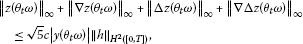(4.2)

where c is the constant in Lemma 2.1.

Lemma 4.1 Let $B={\left\{B\left(\omega \right)\right\}}_{\omega \in \mathrm{\Omega }}\in \mathcal{D}$ and ${\left({u}_{0}\left(\omega \right),{v}_{0}\left(\omega \right)\right)}^{T}\in B\left(\omega \right)$. Then for P-a.e. $\omega \in \mathrm{\Omega }$, there exists ${T}_{B}\left(\omega \right)>0$ and a random variable $r\left(\omega \right)$, such that the solution ${\left(u\left(t,\omega ,{u}_{0}\left(\omega \right)\right),v\left(t,\omega ,{v}_{0}\left(\omega \right)\right)\right)}^{T}$ of (3.13)-(3.17) satisfies, for all $t\ge {T}_{B}\left(\omega \right)$,

$\begin{array}{r}{\parallel u\left(t,{\theta }_{-t}\omega ,{u}_{0}\left({\theta }_{-t}\omega \right)\right)\parallel }_{2}^{2}+{\parallel \mathrm{\nabla }u\left(t,{\theta }_{-t}\omega ,\mathrm{\nabla }{u}_{0}\left({\theta }_{-t}\omega \right)\right)\parallel }_{2}^{2}\\ \phantom{\rule{1em}{0ex}}+{\parallel v\left(t,{\theta }_{-t}\omega ,{v}_{0}\left({\theta }_{-t}\omega \right)\right)\parallel }_{2}^{2}+{\parallel \mathrm{\nabla }v\left(t,{\theta }_{-t}\omega ,\mathrm{\nabla }{v}_{0}\left({\theta }_{-t}\omega \right)\right)\parallel }_{2}^{2}\le r\left(\omega \right).\end{array}$
(4.3)

Proof Taking the inner product of (3.13), (3.14) with u, v in ${L}^{2}\left(\left[0,T\right]\right)$ respectively, we have that(4.4)(4.5)

Integrating by parts, we have(4.6)(4.7)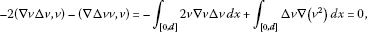(4.8)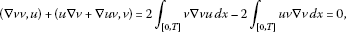(4.9)(4.10)

Associating with (4.6)-(4.10) we note that

$\left({F}_{1}\left(u,v\right),u\right)+\left({F}_{2}\left(u,v\right),v\right)=0.$
(4.11)

Employing Young inequality and Gagliardo-Nirenberg inequality, the terms with random parameter of (4.4) and (4.5) are bounded by(4.12)(4.13)

and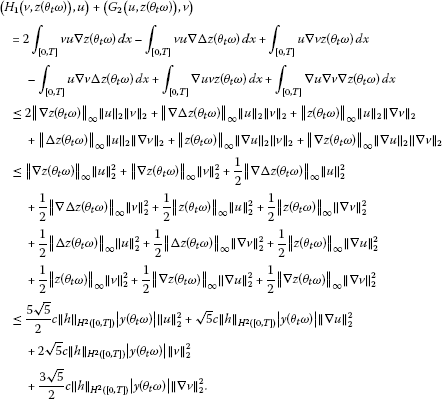(4.14)

According to Poincaré inequality, combining (4.4) with (4.5) and following the estimates from (4.11)-(4.14), we obtain(4.15)

Then (4.15) is equivalent to

$\begin{array}{r}\frac{d}{dt}\left({\parallel u\parallel }_{2}^{2}+{\parallel \mathrm{\nabla }u\parallel }_{2}^{2}+{\parallel v\parallel }_{2}^{2}+{\parallel \mathrm{\nabla }v\parallel }_{2}^{2}\right)+\epsilon \lambda \left({\parallel u\parallel }_{2}^{2}+{\parallel \mathrm{\nabla }u\parallel }_{2}^{2}+{\parallel v\parallel }_{2}^{2}+{\parallel \mathrm{\nabla }v\parallel }_{2}^{2}\right)\\ \phantom{\rule{1em}{0ex}}\le {c}_{2}|y\left({\theta }_{t}\omega \right)|\left({\parallel u\parallel }_{2}^{2}+{\parallel \mathrm{\nabla }u\parallel }_{2}^{2}+{\parallel v\parallel }_{2}^{2}+{\parallel \mathrm{\nabla }v\parallel }_{2}^{2}\right)\\ \phantom{\rule{2em}{0ex}}+{c}_{3}{|y\left({\theta }_{t}\omega \right)|}^{2}\left({\parallel u\parallel }_{2}^{2}+{\parallel \mathrm{\nabla }u\parallel }_{2}^{2}+{\parallel v\parallel }_{2}^{2}+{\parallel \mathrm{\nabla }v\parallel }_{2}^{2}\right)+{c}_{1}{|y\left({\theta }_{t}\omega \right)|}^{2},\end{array}$
(4.16)

where ${c}_{1}=\left(8\epsilon /\lambda +8{\alpha }^{2}/\epsilon \lambda +8\right){\parallel h\parallel }_{{H}^{2}\left(\left[0,T\right]\right)}^{2}$, ${c}_{2}=9\sqrt{5}c{\parallel h\parallel }_{{H}^{2}\left(\left[0,T\right]\right)}$ and ${c}_{3}=30{c}^{2}{\parallel h\parallel }_{{H}^{2}\left(\left[0,T\right]\right)}^{2}$. We denote

$\begin{array}{rl}{N}_{1}\left(t,\omega \right)=& {\parallel u\left(t,\omega ,{u}_{0}\left(\omega \right)\right)\parallel }_{2}^{2}+{\parallel \mathrm{\nabla }u\left(t,\omega ,\mathrm{\nabla }{u}_{0}\left(\omega \right)\right)\parallel }_{2}^{2}\\ +{\parallel v\left(t,\omega ,{v}_{0}\left(\omega \right)\right)\parallel }_{2}^{2}+{\parallel \mathrm{\nabla }v\left(t,\omega ,\mathrm{\nabla }{v}_{0}\left(\omega \right)\right)\parallel }_{2}^{2}.\end{array}$
(4.17)

Applying Gronwall lemma, we obtain that, for all $t\ge 0$,

${N}_{1}\left(t,\omega \right)\le {e}^{J\left(\omega ,0,t\right)}{N}_{1}\left(0,\omega \right)+{c}_{1}{\int }_{0}^{t}{|y\left({\theta }_{s}\omega \right)|}^{2}{e}^{J\left(\omega ,s,t\right)}\phantom{\rule{0.2em}{0ex}}ds,$
(4.18)

where

${J}_{1}\left(\omega ,s,t\right)=\epsilon \lambda s-\epsilon \lambda t+{c}_{2}{\int }_{s}^{t}|y\left({\theta }_{\tau }\omega \right)|\phantom{\rule{0.2em}{0ex}}d\tau +{c}_{3}{\int }_{s}^{t}{|y\left({\theta }_{\tau }\omega \right)|}^{2}\phantom{\rule{0.2em}{0ex}}d\tau .$
(4.19)

Replacing ω by ${\theta }_{-t}\omega$ in (4.18) we obtain that, for all $t\ge 0$,

${N}_{1}\left(t,{\theta }_{-t}\omega \right)\le {e}^{{J}_{1}\left({\theta }_{-t}\omega ,0,t\right)}{N}_{1}\left(0,{\theta }_{-t}\omega \right)+{c}_{1}{\int }_{0}^{t}{|y\left({\theta }_{s-t}\omega \right)|}^{2}{e}^{{J}_{1}\left({\theta }_{-t}\omega ,s,t\right)}\phantom{\rule{0.2em}{0ex}}ds.$
(4.20)

For all $s\in \left(0,t\right)$,

$\begin{array}{rl}{J}_{1}\left({\theta }_{-t}\omega ,s,t\right)=& \epsilon \lambda s-\epsilon \lambda t+{c}_{2}{\int }_{s}^{t}|y\left({\theta }_{\tau -t}\omega \right)|\phantom{\rule{0.2em}{0ex}}d\tau +{c}_{3}{\int }_{s}^{t}{|y\left({\theta }_{\tau -t}\omega \right)|}^{2}\phantom{\rule{0.2em}{0ex}}d\tau \\ =& \epsilon \lambda s-\epsilon \lambda t+{c}_{2}{\int }_{s-t}^{0}|y\left({\theta }_{\tau }\omega \right)|\phantom{\rule{0.2em}{0ex}}d\tau +{c}_{3}{\int }_{s-t}^{0}{|y\left({\theta }_{\tau }\omega \right)|}^{2}\phantom{\rule{0.2em}{0ex}}d\tau \\ =& {J}_{1}\left(\omega ,s-t,0\right).\end{array}$
(4.21)

Then for all $t\ge 0$, (4.20) is equivalent to

${N}_{1}\left(t,{\theta }_{-t}\omega \right)\le {e}^{{J}_{1}\left(\omega ,-t,0\right)}{N}_{1}\left(0,{\theta }_{-t}\omega \right)+{c}_{1}{\int }_{-t}^{0}{|y\left({\theta }_{s}\omega \right)|}^{2}{e}^{{J}_{1}\left(\omega ,s,0\right)}\phantom{\rule{0.2em}{0ex}}ds.$
(4.22)

Lemma 3.1 implies that

$\begin{array}{rcl}\underset{t\to +\mathrm{\infty }}{lim}\frac{{J}_{1}\left(\omega ,-t,0\right)}{t}& =& \underset{t\to +\mathrm{\infty }}{lim}\left(-\epsilon \lambda +\frac{{c}_{2}}{t}{\int }_{-t}^{0}|y\left({\theta }_{\tau }\omega \right)|\phantom{\rule{0.2em}{0ex}}d\tau +\frac{{c}_{3}}{t}{\int }_{-t}^{0}{|y\left({\theta }_{\tau }\omega \right)|}^{2}\phantom{\rule{0.2em}{0ex}}d\tau \right)\\ =& -\epsilon \lambda +\frac{{c}_{2}}{\sqrt{\pi \alpha }}+\frac{{c}_{3}}{2\alpha },\end{array}$
(4.23)

so for P-a.e. $\omega \in \mathrm{\Omega }$, there exists ${T}_{1}\left(\omega \right)>0$ and ${\alpha }_{1}>0$ such that for $t\ge {T}_{1}\left(\omega \right)$ and $\alpha \ge {\alpha }_{1}$,

$-\epsilon \lambda t+{c}_{2}{\int }_{-t}^{0}|y\left({\theta }_{\tau }\omega \right)|\phantom{\rule{0.2em}{0ex}}d\tau +{c}_{3}{\int }_{-t}^{0}{|y\left({\theta }_{\tau }\omega \right)|}^{2}\phantom{\rule{0.2em}{0ex}}d\tau <0.$
(4.24)

Note that $|y\left({\theta }_{t}\omega \right)|$ is tempered, and by (4.23)-(4.24), the integrand of the second term on the right-hand side of (4.22) is convergent to zero exponentially as $s\to -\mathrm{\infty }$. This shows that for P-a.e. $\omega \in \mathrm{\Omega }$, the following integral:

${r}_{1}\left(\omega \right)={c}_{1}{\int }_{-\mathrm{\infty }}^{0}{|y\left({\theta }_{s}\omega \right)|}^{2}{e}^{{J}_{1}\left(\omega ,s,0\right)}\phantom{\rule{0.2em}{0ex}}ds$
(4.25)

is convergent. By assumption, ${\left\{B\left(\omega \right)\right\}}_{\omega \in \mathrm{\Omega }}\in \mathcal{D}$ is tempered. Therefore, if ${\left({u}_{0}\left({\theta }_{-t}\omega \right),{v}_{0}\left({\theta }_{-t}\omega \right)\right)}^{T}\in B\left({\theta }_{-t}\omega \right)$, then there is ${T}_{2}={T}_{2}\left(B,\omega \right)\ge {T}_{1}\left(\omega \right)$ such that for all $t\ge {T}_{2}$,

${e}^{{J}_{1}\left(\omega ,-t,0\right)}{N}_{1}\left(0,{\theta }_{-t}\omega \right)\le {r}_{1}\left(\omega \right).$
(4.26)

Let ${r}_{2}\left(\omega \right)=2{r}_{1}\left(\omega \right)$, we get that for all $t\ge {T}_{2}\left(\omega \right)$,

${N}_{1}\left(t,{\theta }_{-t}\omega \right)\le {r}_{2}\left(\omega \right),$
(4.27)

which completes the proof. □

Lemma 4.2 Let $B={\left\{B\left(\omega \right)\right\}}_{\omega \in \mathrm{\Omega }}\in \mathcal{D}$ and ${\left({u}_{0}\left(\omega \right),{v}_{0}\left(\omega \right)\right)}^{T}\in B\left(\omega \right)$. Then for P-a.e. $\omega \in \mathrm{\Omega }$, there exists ${T}_{B}\left(\omega \right)>0$ and a random variable $r\left(\omega \right)$, such that the solutions ${\left(u\left(t,\omega ,{u}_{0}\left(\omega \right)\right),v\left(t,\omega ,{v}_{0}\left(\omega \right)\right)\right)}^{T}$ of (3.13)-(3.17) satisfy, for all $t\ge {T}_{B}\left(\omega \right)$,

$\begin{array}{r}{\int }_{t}^{t+1}\left({\parallel \mathrm{\nabla }u\left(s,{\theta }_{-t-1}\omega ,\mathrm{\nabla }{u}_{0}\left({\theta }_{-t-1}\omega \right)\right)\parallel }_{2}^{2}+{\parallel \mathrm{\Delta }u\left(s,{\theta }_{-t-1}\omega ,\mathrm{\Delta }{u}_{0}\left({\theta }_{-t-1}\omega \right)\right)\parallel }_{2}^{2}\\ \phantom{\rule{2em}{0ex}}+{\parallel \mathrm{\nabla }v\left(s,{\theta }_{-t-1}\omega ,\mathrm{\nabla }{v}_{0}\left({\theta }_{-t-1}\omega \right)\right)\parallel }_{2}^{2}+{\parallel \mathrm{\Delta }v\left(s,{\theta }_{-t-1}\omega ,\mathrm{\Delta }{v}_{0}\left({\theta }_{-t-1}\omega \right)\right)\parallel }_{2}^{2}\right)\phantom{\rule{0.2em}{0ex}}ds\\ \phantom{\rule{1em}{0ex}}\le r\left(\omega \right).\end{array}$
(4.28)

Proof Now we denote

$\begin{array}{rcl}{N}_{2}\left(t,\omega \right)& =& {\parallel \mathrm{\nabla }u\left(t,\omega ,\mathrm{\nabla }{u}_{0}\left(\omega \right)\right)\parallel }_{2}^{2}+{\parallel \mathrm{\Delta }u\left(t,\omega ,\mathrm{\Delta }{u}_{0}\left(\omega \right)\right)\parallel }_{2}^{2}\\ +{\parallel \mathrm{\nabla }v\left(t,\omega ,\mathrm{\nabla }{v}_{0}\left(\omega \right)\right)\parallel }_{2}^{2}+{\parallel \mathrm{\Delta }v\left(t,\omega ,\mathrm{\Delta }{v}_{0}\left(\omega \right)\right)\parallel }_{2}^{2}.\end{array}$
(4.29)

From the statements and estimates above, we get that

$\begin{array}{rcl}\frac{d}{dt}{N}_{1}\left(t,\omega \right)+2\epsilon {N}_{2}\left(t,\omega \right)& \le & \epsilon \lambda {N}_{1}\left(t,\omega \right)+{c}_{2}|y\left({\theta }_{t}\omega \right)|{N}_{1}\left(t,\omega \right)\\ +{c}_{3}{|y\left({\theta }_{t}\omega \right)|}^{2}{N}_{1}\left(t,\omega \right)+{c}_{1}{|y\left({\theta }_{t}\omega \right)|}^{2}.\end{array}$
(4.30)

Integrating (4.30) with respect to s over $\left[t,t+1\right]$, we get that

$\begin{array}{rcl}{\int }_{t}^{t+1}{N}_{2}\left(s,\omega \right)\phantom{\rule{0.2em}{0ex}}ds& \le & \frac{1}{2\epsilon }{\int }_{t}^{t+1}\left(\epsilon \lambda +{c}_{2}|y\left({\theta }_{s}\omega \right)|+{c}_{3}{|y\left({\theta }_{s}\omega \right)|}^{2}\right){N}_{1}\left(s,\omega \right)\phantom{\rule{0.2em}{0ex}}ds\\ +\frac{1}{2\epsilon }{N}_{1}\left(t,\omega \right)+\frac{{c}_{1}}{2\epsilon }{\int }_{t}^{t+1}{|y\left({\theta }_{s}\omega \right)|}^{2}\phantom{\rule{0.2em}{0ex}}ds.\end{array}$
(4.31)

Replacing ω by ${\theta }_{-t-1}\omega$ in (4.31), we get

$\begin{array}{rcl}{\int }_{t}^{t+1}{N}_{2}\left(s,{\theta }_{-t-1}\omega \right)\phantom{\rule{0.2em}{0ex}}ds& \le & \frac{1}{2\epsilon }{\int }_{t}^{t+1}\left(\epsilon \lambda +{c}_{2}|y\left({\theta }_{s-t-1}\omega \right)|+{c}_{3}{|y\left({\theta }_{s-t-1}\omega \right)|}^{2}\right){N}_{1}\left(s,{\theta }_{-t-1}\omega \right)\phantom{\rule{0.2em}{0ex}}ds\\ +\frac{1}{2\epsilon }{N}_{1}\left(t,{\theta }_{-t-1}\omega \right)+\frac{{c}_{1}}{2\epsilon }{\int }_{t}^{t+1}{|y\left({\theta }_{s-t-1}\omega \right)|}^{2}\phantom{\rule{0.2em}{0ex}}ds.\end{array}$
(4.32)

Replacing t by s, where $s\in \left[t,t+1\right]$, and then replacing ω by ${\theta }_{-t-1}\omega$ in (4.18), we get that

${N}_{1}\left(s,{\theta }_{-t-1}\omega \right)\le {e}^{{J}_{1}\left({\theta }_{-t-1}\omega ,0,s\right)}{N}_{1}\left(0,{\theta }_{-t-1}\omega \right)+{c}_{1}{\int }_{0}^{s}{|y\left({\theta }_{\tau -t-1}\omega \right)|}^{2}{e}^{{J}_{1}\left({\theta }_{-t-1}\omega ,\tau ,s\right)}\phantom{\rule{0.2em}{0ex}}d\tau .$
(4.33)

As the discussion in (4.21), for all $\tau \in \left(0,s\right)$, we have

$\begin{array}{rcl}{J}_{1}\left({\theta }_{-t-1}\omega ,\tau ,s\right)& =& \epsilon \lambda \tau -\epsilon \lambda s+{c}_{2}{\int }_{\tau }^{s}|y\left({\theta }_{\sigma -t-1}\omega \right)|\phantom{\rule{0.2em}{0ex}}d\sigma +{c}_{3}{\int }_{\tau }^{s}{|y\left({\theta }_{\sigma -t-1}\omega \right)|}^{2}\phantom{\rule{0.2em}{0ex}}d\sigma \\ =& \epsilon \lambda \tau -\epsilon \lambda s+{c}_{2}{\int }_{\tau -t-1}^{s-t-1}|y\left({\theta }_{\sigma }\omega \right)|\phantom{\rule{0.2em}{0ex}}d\sigma +{c}_{3}{\int }_{\tau -t-1}^{s-t-1}{|y\left({\theta }_{\sigma }\omega \right)|}^{2}\phantom{\rule{0.2em}{0ex}}d\sigma \\ \le & \epsilon \lambda \tau -\epsilon \lambda t+{c}_{2}{\int }_{\tau -t-1}^{0}|y\left({\theta }_{\sigma }\omega \right)|\phantom{\rule{0.2em}{0ex}}d\sigma +{c}_{3}{\int }_{\tau -t-1}^{0}{|y\left({\theta }_{\sigma }\omega \right)|}^{2}\phantom{\rule{0.2em}{0ex}}d\sigma \\ =& \epsilon \lambda \left(\tau -t-1\right)+{c}_{2}{\int }_{\tau -t-1}^{0}|y\left({\theta }_{\sigma }\omega \right)|\phantom{\rule{0.2em}{0ex}}d\sigma +{c}_{3}{\int }_{\tau -t-1}^{0}{|y\left({\theta }_{\sigma }\omega \right)|}^{2}\phantom{\rule{0.2em}{0ex}}d\sigma +\epsilon \lambda \\ =& {J}_{1}\left(\omega ,\tau -t-1,0\right)+\epsilon \lambda \end{array}$
(4.34)

which states

${N}_{1}\left(s,{\theta }_{-t-1}\omega \right)\le {e}^{\epsilon \lambda }{e}^{{J}_{1}\left(\omega ,-t-1,0\right)}{N}_{1}\left(0,{\theta }_{-t-1}\omega \right)+{c}_{1}{e}^{\epsilon \lambda }{\int }_{-t-1}^{0}{|y\left({\theta }_{\tau }\omega \right)|}^{2}{e}^{{J}_{1}\left(\omega ,\tau ,0\right)}\phantom{\rule{0.2em}{0ex}}d\tau .$
(4.35)

As the consideration from (4.23)-(4.27), which implies that when $t\ge {T}_{2}\left(\omega \right)$, $s\in \left[t,t+1\right]$,

${N}_{1}\left(s,{\theta }_{-t-1}\omega \right)\le {r}_{3}\left(\omega \right),$
(4.36)

where ${r}_{3}={e}^{\epsilon \lambda }{r}_{2}\left(\omega \right)$. Then

$\begin{array}{rcl}{\int }_{t}^{t+1}{N}_{2}\left(s,{\theta }_{-t-1}\omega \right)\phantom{\rule{0.2em}{0ex}}ds& \le & \frac{1}{2\epsilon }{r}_{3}\left(\omega \right)+\frac{\lambda }{2}{r}_{3}\left(\omega \right)+\frac{{c}_{2}}{2\epsilon }{r}_{3}\left(\omega \right){\int }_{t}^{t+1}|y\left({\theta }_{s-t-1}\omega \right)|\phantom{\rule{0.2em}{0ex}}ds\\ +\left(\frac{{c}_{3}}{2\epsilon }{r}_{3}\left(\omega \right)+\frac{{c}_{1}}{2\epsilon }\right){\int }_{t}^{t+1}{|y\left({\theta }_{s-t-1}\omega \right)|}^{2}\phantom{\rule{0.2em}{0ex}}ds\\ \le & \frac{1}{2\epsilon }{r}_{3}\left(\omega \right)+\frac{\lambda }{2}{r}_{3}\left(\omega \right)+\frac{{c}_{2}}{2\epsilon }{r}_{3}\left(\omega \right){\int }_{-1}^{0}|y\left({\theta }_{s}\omega \right)|\phantom{\rule{0.2em}{0ex}}ds\\ +\left(\frac{{c}_{3}}{2\epsilon }{r}_{3}\left(\omega \right)+\frac{{c}_{1}}{2\epsilon }\right){\int }_{-1}^{0}{|y\left({\theta }_{s}\omega \right)|}^{2}\phantom{\rule{0.2em}{0ex}}ds.\end{array}$
(4.37)

Following from Proposition 4.3.3 in , there exists a tempered function ${r}_{4}\left(\omega \right)>0$ such that

$|y\left(\omega \right)|+{|y\left(\omega \right)|}^{2}\le {r}_{4}\left(\omega \right),$
(4.38)

where ${r}_{4}\left(\omega \right)$ satisfies, for P-a.e. $\omega \in \mathrm{\Omega }$,

${r}_{4}\left({\theta }_{t}\omega \right)\le {e}^{\frac{\alpha }{2}|t|}{r}_{4}\left(\omega \right),\phantom{\rule{1em}{0ex}}t\in \mathbb{R}.$
(4.39)

Along with (4.38)-(4.39), we conclude that

${\int }_{t}^{t+1}{N}_{2}\left(s,{\theta }_{-t-1}\omega \right)\phantom{\rule{0.2em}{0ex}}ds\le {r}_{5}\left(\omega \right),$
(4.40)

where

${r}_{5}\left(\omega \right)=\frac{1}{2\epsilon }{r}_{3}\left(\omega \right)+\frac{\lambda }{2}{r}_{3}\left(\omega \right)+\frac{{c}_{2}}{\epsilon \alpha }{e}^{\frac{\alpha }{2}}{r}_{3}\left(\omega \right){r}_{4}\left(\omega \right)+\frac{{c}_{3}}{\epsilon \alpha }{e}^{\frac{\alpha }{2}}{r}_{3}\left(\omega \right){r}_{4}\left(\omega \right)+\frac{{c}_{1}}{\alpha \epsilon }{e}^{\frac{\alpha }{2}}{r}_{4}\left(\omega \right),$
(4.41)

which completes the proof. □

Lemma 4.3 Let $B={\left\{B\left(\omega \right)\right\}}_{\omega \in \mathrm{\Omega }}\in \mathcal{D}$ and ${\left({u}_{0}\left(\omega \right),{v}_{0}\left(\omega \right)\right)}^{T}\in B\left(\omega \right)$. Then for P-a.e. $\omega \in \mathrm{\Omega }$, there exists ${T}_{B}\left(\omega \right)>0$ and a random variable $r\left(\omega \right)$, such that the solutions ${\left(u\left(t,\omega ,{u}_{0}\left(\omega \right)\right),v\left(t,\omega ,{v}_{0}\left(\omega \right)\right)\right)}^{T}$ of (3.13)-(3.17) satisfy, for all $t\ge {T}_{B}\left(\omega \right)$,

${\parallel \mathrm{\Delta }u\left(t,{\theta }_{-t}\omega ,\mathrm{\Delta }{u}_{0}\left({\theta }_{-t}\omega \right)\right)\parallel }_{2}^{2}+{\parallel \mathrm{\Delta }v\left(t,{\theta }_{-t}\omega ,\mathrm{\Delta }{v}_{0}\left({\theta }_{-t}\omega \right)\right)\parallel }_{2}^{2}\le r\left(\omega \right).$
(4.42)

Proof Taking the inner product of (3.13) and (3.14) in ${L}^{2}\left(\left[0,T\right]\right)$ respectively with $-\mathrm{\Delta }u$ and $-\mathrm{\Delta }v$, we have(4.43)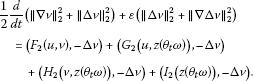(4.44)

By integrating by parts, we get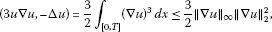(4.45)(4.46)(4.47)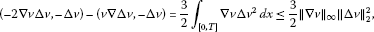(4.48)(4.49)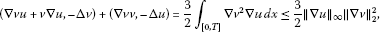(4.50)(4.51)

Employing Young inequality and Gagliardo-Nirenberg inequality, along with (4.45)-(4.51), we get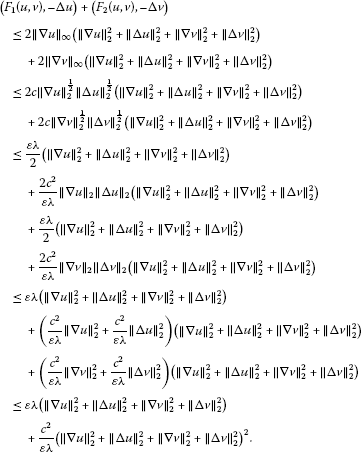(4.52)

Then we estimate the terms with random parameter. They are bounded by(4.53)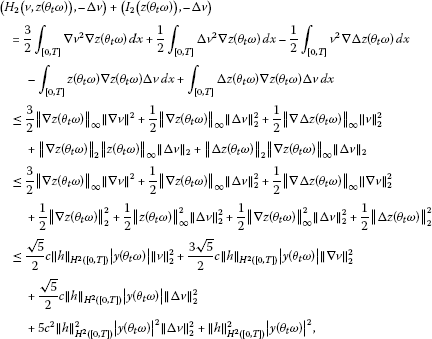(4.54)

and(4.55)

According to Poincaré inequality along with (4.52)-(4.55), we obtain

$\begin{array}{rl}\frac{d}{dt}{N}_{2}\left(t,\omega \right)\le & {c}_{4}{N}_{2}{\left(t,\omega \right)}^{2}+{c}_{5}{N}_{2}\left(t,\omega \right)+{c}_{6}|y\left({\theta }_{t}\omega \right)|{N}_{1}\left(t,\omega \right)\\ +{c}_{7}|y\left({\theta }_{t}\omega \right)|{N}_{2}\left(t,\omega \right)+{c}_{8}{|y\left({\theta }_{t}\omega \right)|}^{2}{N}_{2}\left(t,\omega \right)+{c}_{9}{|y\left({\theta }_{t}\omega \right)|}^{2},\end{array}$
(4.56)

where ${c}_{4}=2{c}^{2}/\epsilon \lambda$, ${c}_{5}=2\epsilon +2\alpha$, ${c}_{6}=5\sqrt{5}c{\parallel h\parallel }_{{H}^{2}\left(\left[0,T\right]\right)}$, ${c}_{7}=14\sqrt{5}c{\parallel h\parallel }_{{H}^{2}\left(\left[0,T\right]\right)}$ and ${c}_{8}=30{c}^{2}{\parallel h\parallel }_{{H}^{2}\left(\left[0,T\right]\right)}^{2}$, ${c}_{9}=\left(2\epsilon +2\alpha +8\right){\parallel h\parallel }_{{H}^{2}\left(\left[0,T\right]\right)}^{2}$. Applying Gronwall lemma over $\left[s,t+1\right]$, where $s\in \left[t,t+1\right]$ and $t\ge {T}_{2}\left(\omega \right)$, we get

$\begin{array}{rcl}{N}_{2}\left(t+1,\omega \right)& \le & {e}^{{J}_{2}\left(\omega ,s,t+1\right)}{N}_{2}\left(s,\omega \right)+{c}_{9}{\int }_{s}^{t+1}{|y\left({\theta }_{\tau }\omega \right)|}^{2}{e}^{{J}_{2}\left(\omega ,\tau ,t+1\right)}\phantom{\rule{0.2em}{0ex}}d\tau \\ +{c}_{6}{\int }_{s}^{t+1}|y\left({\theta }_{\tau }\omega \right)|{N}_{1}\left(\tau ,\omega \right){e}^{{J}_{2}\left(\omega ,\tau ,t+1\right)}\phantom{\rule{0.2em}{0ex}}d\tau ,\end{array}$
(4.57)

where

${J}_{2}\left(\omega ,s,t\right)={c}_{4}{\int }_{s}^{t}{N}_{2}\left(\tau ,\omega \right)\phantom{\rule{0.2em}{0ex}}d\tau +{\int }_{s}^{t}\left({c}_{5}+{c}_{7}|y\left({\theta }_{\tau }\omega \right)|+{c}_{8}{|y\left({\theta }_{\tau }\omega \right)|}^{2}\right)\phantom{\rule{0.2em}{0ex}}d\tau .$
(4.58)

Replacing ω by ${\theta }_{-t-1}\omega$ in (4.57), we obtain that

$\begin{array}{rcl}{N}_{2}\left(t+1,{\theta }_{-t-1}\omega \right)& \le & {e}^{{J}_{2}\left({\theta }_{-t-1}\omega ,s,t+1\right)}{N}_{2}\left(s,{\theta }_{-t-1}\omega \right)+{c}_{9}{\int }_{s}^{t+1}{|y\left({\theta }_{\tau -t-1}\omega \right)|}^{2}{e}^{{J}_{2}\left({\theta }_{-t-1}\omega ,\tau ,t+1\right)}\phantom{\rule{0.2em}{0ex}}d\tau \\ +{c}_{6}{\int }_{s}^{t+1}|y\left({\theta }_{\tau -t-1}\omega \right)|{N}_{1}\left(\tau ,{\theta }_{-t-1}\omega \right){e}^{{J}_{2}\left({\theta }_{-t-1}\omega ,\tau ,t+1\right)}\phantom{\rule{0.2em}{0ex}}d\tau .\end{array}$
(4.59)

For $s\in \left(t,t+1\right)$ and $t\ge {T}_{2}\left(\omega \right)$, we have

$\begin{array}{r}{J}_{2}\left({\theta }_{-t-1}\omega ,s,t+1\right)\\ \phantom{\rule{1em}{0ex}}={c}_{4}{\int }_{s}^{t+1}{N}_{2}\left(\tau ,{\theta }_{-t-1}\omega \right)\phantom{\rule{0.2em}{0ex}}d\tau +{\int }_{s}^{t+1}\left({c}_{5}+{c}_{7}|y\left({\theta }_{\tau -t-1}\omega \right)|+{c}_{8}{|y\left({\theta }_{\tau -t-1}\omega \right)|}^{2}\right)\phantom{\rule{0.2em}{0ex}}d\tau \\ \phantom{\rule{1em}{0ex}}\le {c}_{4}{\int }_{t}^{t+1}{N}_{2}\left(\tau ,{\theta }_{-t-1}\omega \right)\phantom{\rule{0.2em}{0ex}}d\tau +{\int }_{t}^{t+1}\left({c}_{5}+{c}_{7}|y\left({\theta }_{\tau -t-1}\omega \right)|+{c}_{8}{|y\left({\theta }_{\tau -t-1}\omega \right)|}^{2}\right)\phantom{\rule{0.2em}{0ex}}d\tau \\ \phantom{\rule{1em}{0ex}}\le {c}_{4}{r}_{5}\left(\omega \right)+{c}_{5}+{c}_{7}{\int }_{-1}^{0}|y\left({\theta }_{\tau }\omega \right)|\phantom{\rule{0.2em}{0ex}}d\tau +{c}_{8}{\int }_{-1}^{0}{|y\left({\theta }_{\tau }\omega \right)|}^{2}\phantom{\rule{0.2em}{0ex}}d\tau \\ \phantom{\rule{1em}{0ex}}\le {c}_{4}{r}_{5}\left(\omega \right)+{c}_{5}+{c}_{7}{r}_{4}\left(\omega \right){\int }_{-1}^{0}{e}^{-\frac{\alpha \tau }{2}}\phantom{\rule{0.2em}{0ex}}d\tau +{c}_{8}{r}_{4}\left(\omega \right){\int }_{-1}^{0}{e}^{-\frac{\alpha \tau }{2}}\phantom{\rule{0.2em}{0ex}}d\tau \\ \phantom{\rule{1em}{0ex}}\le {c}_{4}{r}_{5}\left(\omega \right)+{c}_{5}+\frac{2{c}_{7}}{\alpha }{e}^{\frac{\alpha }{2}}{r}_{4}\left(\omega \right)+\frac{2{c}_{8}}{\alpha }{e}^{\frac{\alpha }{2}}{r}_{4}\left(\omega \right).\end{array}$
(4.60)

We denote

${r}_{6}\left(\omega \right)={c}_{4}{r}_{5}\left(\omega \right)+{c}_{5}+\frac{2{c}_{7}}{\alpha }{e}^{\frac{\alpha }{2}}{r}_{4}\left(\omega \right)+\frac{2{c}_{8}}{\alpha }{e}^{\frac{\alpha }{2}}{r}_{4}\left(\omega \right),$
(4.61)

then along with (4.36) we obtain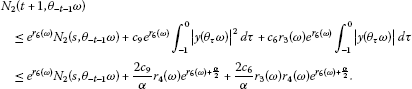(4.62)

Now integrating (4.62) with respect to s over $\left(t,t+1\right)$ where $t\ge {T}_{2}\left(\omega \right)$, we obtain that(4.63)

Associating with Lemma 4.2, we see that

${N}_{2}\left(t+1,{\theta }_{-t-1}\omega \right)\le {r}_{7}\left(\omega \right),$
(4.64)

where

${r}_{7}\left(\omega \right)={e}^{{r}_{6}\left(\omega \right)}{r}_{5}\left(\omega \right)+\frac{2{c}_{9}}{\alpha }{r}_{4}\left(\omega \right){e}^{{r}_{6}\left(\omega \right)+\frac{\alpha }{2}}+\frac{2{c}_{6}}{\alpha }{r}_{3}\left(\omega \right){r}_{4}\left(\omega \right){e}^{{r}_{6}\left(\omega \right)+\frac{\alpha }{2}},$
(4.65)

which completes the proof. □

So far, Lemma 4.1 and Lemma 4.3 implies that there exists a random variable $r\left(\omega \right)$ such that for all $t\ge {T}_{2}\left(\omega \right)$,

${\parallel u\left(t,{\theta }_{-t}\omega ,{u}_{0}\left({\theta }_{-t}\omega \right)\right)\parallel }_{{H}^{2}\left(\left[0,T\right]\right)}^{2}+{\parallel v\left(t,{\theta }_{-t}\omega ,{v}_{0}\left({\theta }_{-t}\omega \right)\right)\parallel }_{{H}^{2}\left(\left[0,T\right]\right)}^{2}\le r\left(\omega \right).$
(4.66)

The next lemma will illustrate the existence of the random absorbing set for Φ in $\mathcal{D}$.

Lemma 4.4 There exists ${\left\{K\left(\omega \right)\right\}}_{\omega \in \mathrm{\Omega }}\in \mathcal{D}$ such that ${\left\{K\left(\omega \right)\right\}}_{\omega \in \mathrm{\Omega }}$ is a random absorbing set for Φ in $\mathcal{D}$, that is, for any $B={\left\{B\left(\omega \right)\right\}}_{\omega \in \mathrm{\Omega }}\in \mathcal{D}$ and P-a.e. $\omega \in \mathrm{\Omega }$, there is ${T}_{B}>0$ such that

$\mathrm{\Phi }\left(t,{\theta }_{-t}\omega ,B\left({\theta }_{-t}\omega \right)\right)\subseteq K\left(\omega \right),\phantom{\rule{1em}{0ex}}\mathrm{\forall }t\ge {T}_{B}\left(\omega \right).$

Proof We denote

$K\left(\omega \right)=\left\{{\left(u,v\right)}^{T}\in {H}^{2}\left(\left[0,T\right]\right)×{H}^{2}\left(\left[0,T\right]\right):{\parallel u\parallel }_{{H}^{2}\left(\left[0,T\right]\right)}^{2}+{\parallel v\parallel }_{{H}^{2}\left(\left[0,T\right]\right)}^{2}\le r\left(\omega \right)\right\}.$
(4.67)

Then ${\left\{K\left(\omega \right)\right\}}_{\omega \in \mathrm{\Omega }}\in \mathcal{D}$. Further, (4.67) indicates that ${\left\{K\left(\omega \right)\right\}}_{\omega \in \mathrm{\Omega }}$ is a random absorbing set for Φ in $\mathcal{D}$, which completes the proof. □

Next we derive uniform estimates for ${\left(u,v\right)}^{T}$ in ${H}^{3}\left(\left[0,T\right]\right)×{H}^{3}\left(\left[0,T\right]\right)$.

Lemma 4.5 Let $B={\left\{B\left(\omega \right)\right\}}_{\omega \in \mathrm{\Omega }}\in \mathcal{D}$ and ${\left({u}_{0}\left(\omega \right),{v}_{0}\left(\omega \right)\right)}^{T}\in B\left(\omega \right)$. Then for P-a.e. $\omega \in \mathrm{\Omega }$, there exists ${T}_{B}\left(\omega \right)>0$ and a random variable $r\left(\omega \right)$, such that the solutions ${\left(u\left(t,\omega ,{u}_{0}\left(\omega \right)\right),v\left(t,\omega ,{v}_{0}\left(\omega \right)\right)\right)}^{T}$ of (3.13)-(3.17) satisfy, for all $t\ge {T}_{B}\left(\omega \right)$,

$\begin{array}{r}{\int }_{t}^{t+1}\left({\parallel \mathrm{\Delta }u\left(s,{\theta }_{-t-1}\omega ,\mathrm{\Delta }{u}_{0}\left({\theta }_{-t-1}\omega \right)\right)\parallel }_{2}^{2}+{\parallel \mathrm{\nabla }\mathrm{\Delta }u\left(s,{\theta }_{-t-1}\omega ,\mathrm{\nabla }\mathrm{\Delta }{u}_{0}\left({\theta }_{-t-1}\omega \right)\right)\parallel }_{2}^{2}\\ \phantom{\rule{2em}{0ex}}+{\parallel \mathrm{\Delta }v\left(s,{\theta }_{-t-1}\omega ,\mathrm{\Delta }{v}_{0}\left({\theta }_{-t-1}\omega \right)\right)\parallel }_{2}^{2}+{\parallel \mathrm{\nabla }\mathrm{\Delta }v\left(s,{\theta }_{-t-1}\omega ,\mathrm{\nabla }\mathrm{\Delta }{v}_{0}\left({\theta }_{-t-1}\omega \right)\right)\parallel }_{2}^{2}\right)\phantom{\rule{0.2em}{0ex}}ds\\ \phantom{\rule{1em}{0ex}}\le r\left(\omega \right).\end{array}$
(4.68)

Proof Now we denote

$\begin{array}{rcl}{N}_{3}\left(t,\omega \right)& =& {\parallel \mathrm{\Delta }u\left(t,\omega ,\mathrm{\Delta }{u}_{0}\left(\omega \right)\right)\parallel }_{2}^{2}+{\parallel \mathrm{\Delta }\mathrm{\nabla }u\left(t,\omega ,\mathrm{\Delta }{u}_{0}\left(\omega \right)\right)\parallel }_{2}^{2}\\ +{\parallel \mathrm{\Delta }v\left(t,\omega ,\mathrm{\Delta }{u}_{0}\left(\omega \right)\right)\parallel }_{2}^{2}+{\parallel \mathrm{\Delta }\mathrm{\nabla }v\left(t,\omega ,\mathrm{\Delta }{u}_{0}\left(\omega \right)\right)\parallel }_{2}^{2}.\end{array}$
(4.69)

Associating with (4.43)-(4.44) and (4.52)-(4.55), we get

$\begin{array}{r}\frac{d}{dt}{N}_{2}\left(t,\omega \right)+2\epsilon {N}_{3}\left(t,\omega \right)\\ \phantom{\rule{1em}{0ex}}\le {c}_{4}{N}_{2}{\left(t,\omega \right)}^{2}+{c}_{10}{N}_{2}\left(t,\omega \right)+{c}_{6}|y\left({\theta }_{t}\omega \right)|{N}_{1}\left(t,\omega \right)\\ \phantom{\rule{2em}{0ex}}+{c}_{7}|y\left({\theta }_{t}\omega \right)|{N}_{2}\left(t,\omega \right)+{c}_{8}{|y\left({\theta }_{t}\omega \right)|}^{2}{N}_{2}\left(t,\omega \right)+{c}_{9}{|y\left({\theta }_{t}\omega \right)|}^{2},\end{array}$
(4.70)

where ${c}_{10}=2\epsilon \lambda +{c}_{5}$. Applying Gronwall lemma over $\left[t,t+1\right]$ where $t\ge {T}_{2}\left(\omega \right)$, then we obtain

$\begin{array}{r}{N}_{2}\left(t+1,\omega \right)+2\epsilon {\int }_{t}^{t+1}{N}_{3}\left(s,\omega \right){e}^{{J}_{3}\left(\omega ,s,t+1\right)}\phantom{\rule{0.2em}{0ex}}ds\\ \phantom{\rule{1em}{0ex}}\le {e}^{{J}_{3}\left(\omega ,t,t+1\right)}{N}_{2}\left(t,\omega \right)+{c}_{9}{\int }_{t}^{t+1}{|y\left({\theta }_{s}\omega \right)|}^{2}{e}^{{J}_{3}\left(\omega ,s,t+1\right)}\phantom{\rule{0.2em}{0ex}}ds\\ \phantom{\rule{2em}{0ex}}+{c}_{6}{\int }_{t}^{t+1}|y\left({\theta }_{s}\omega \right)|{N}_{1}\left(s,\omega \right){e}^{{J}_{3}\left(\omega ,s,t+1\right)}\phantom{\rule{0.2em}{0ex}}ds,\end{array}$
(4.71)

where

${J}_{3}\left(\omega ,s,t\right)={c}_{4}{\int }_{s}^{t}{N}_{2}\left(\tau ,\omega \right)\phantom{\rule{0.2em}{0ex}}d\tau +{\int }_{s}^{t}\left({c}_{10}+{c}_{7}|y\left({\theta }_{\tau }\omega \right)|+{c}_{8}{|y\left({\theta }_{\tau }\omega \right)|}^{2}\right)\phantom{\rule{0.2em}{0ex}}d\tau .$
(4.72)

Replacing ω by ${\theta }_{-t-1}\omega$ in (4.71), we get

$\begin{array}{rcl}{N}_{2}\left(t+1,{\theta }_{-t-1}\omega \right)& \le & {e}^{{J}_{3}\left({\theta }_{-t-1}\omega ,t,t+1\right)}{N}_{2}\left(t,{\theta }_{-t-1}\omega \right)+{c}_{9}{\int }_{t}^{t+1}{|y\left({\theta }_{s-t-1}\omega \right)|}^{2}{e}^{{J}_{3}\left({\theta }_{-t-1}\omega ,s,t+1\right)}\phantom{\rule{0.2em}{0ex}}ds\\ +{c}_{6}{\int }_{t}^{t+1}|y\left({\theta }_{s-t-1}\omega \right)|{N}_{1}\left(s,{\theta }_{-t-1}\omega \right){e}^{{J}_{3}\left({\theta }_{-t-1}\omega ,s,t+1\right)}\phantom{\rule{0.2em}{0ex}}ds\\ -2\epsilon {\int }_{t}^{t+1}\left({N}_{3}\left(s,{\theta }_{-t-1}\omega \right)\right){e}^{{J}_{3}\left({\theta }_{-t-1}\omega ,s,t\right)}\phantom{\rule{0.2em}{0ex}}ds.\end{array}$
(4.73)

As the discussion in (4.21)-(4.27), for $s\in \left[t,t+1\right]$ and $t\ge {T}_{2}\left(\omega \right)$, we have

${N}_{1}\left(s,{\theta }_{-t-2}\omega \right)\le {e}^{2\epsilon \lambda }{r}_{2}\left(\omega \right).$
(4.74)

Replacing ω by ${\theta }_{-t-2}\omega$ in (4.31), we obtain that

$\begin{array}{r}{\int }_{s}^{t+1}{N}_{2}\left(\tau ,{\theta }_{-t-2}\omega \right)\phantom{\rule{0.2em}{0ex}}d\tau \\ \phantom{\rule{1em}{0ex}}\le \frac{1}{2\epsilon }{\int }_{t}^{t+1}\left(\epsilon \lambda +{c}_{2}|y\left({\theta }_{\tau -t-2}\omega \right)|+{c}_{3}{|y\left({\theta }_{\tau -t-2}\omega \right)|}^{2}\right){N}_{1}\left(\tau ,{\theta }_{-t-2}\omega \right)\phantom{\rule{0.2em}{0ex}}d\tau \\ \phantom{\rule{2em}{0ex}}+\frac{1}{2\epsilon }{N}_{1}\left(s,{\theta }_{-t-2}\omega \right)+\frac{{c}_{1}}{2\epsilon }{\int }_{t}^{t+1}{|y\left({\theta }_{\tau -t-2}\omega \right)|}^{2}\phantom{\rule{0.2em}{0ex}}d\tau \\ \phantom{\rule{1em}{0ex}}\le \frac{1}{2\epsilon }{e}^{2\epsilon \lambda }{r}_{2}\left(\omega \right){\int }_{-2}^{0}\left(\epsilon \lambda +{c}_{2}|y\left({\theta }_{\tau }\omega \right)|+{c}_{3}{|y\left({\theta }_{\tau }\omega \right)|}^{2}\right)\phantom{\rule{0.2em}{0ex}}d\tau +\frac{1}{2\epsilon }{e}^{2\epsilon \lambda }{r}_{2}\left(\omega \right)\\ \phantom{\rule{2em}{0ex}}+\frac{{c}_{1}}{2\epsilon }{\int }_{-2}^{0}{|y\left({\theta }_{\tau }\omega \right)|}^{2}\phantom{\rule{0.2em}{0ex}}d\tau \\ \phantom{\rule{1em}{0ex}}\le \frac{1}{2\epsilon }{e}^{2\epsilon \lambda }{r}_{2}\left(\omega \right){\int }_{-2}^{0}\left(\epsilon \lambda +{c}_{2}{e}^{-\frac{\alpha }{2}\tau }{r}_{4}\left(\omega \right)+{c}_{3}{e}^{-\frac{\alpha }{2}\tau }{r}_{4}\left(\omega \right)\right)\phantom{\rule{0.2em}{0ex}}d\tau +\frac{1}{2\epsilon }{e}^{2\epsilon \lambda }{r}_{2}\left(\omega \right)\\ \phantom{\rule{2em}{0ex}}+\frac{{c}_{1}}{2\epsilon }{r}_{4}\left(\omega \right){\int }_{-2}^{0}{e}^{-\frac{\alpha }{2}\tau }\phantom{\rule{0.2em}{0ex}}d\tau \\ \phantom{\rule{1em}{0ex}}\le {r}_{8}\left(\omega \right),\end{array}$
(4.75)

where

$\begin{array}{rcl}{r}_{8}\left(\omega \right)& =& \frac{1}{2\epsilon }{e}^{2\epsilon \lambda }{r}_{2}\left(\omega \right)\left(2\epsilon \lambda +\frac{2{c}_{2}}{\alpha }{e}^{\alpha }{r}_{4}\left(\omega \right)+\frac{2{c}_{3}}{\alpha }{e}^{\alpha }{r}_{4}\left(\omega \right)\right)\\ +\frac{1}{2\epsilon }{e}^{2\epsilon \lambda }{r}_{2}\left(\omega \right)+\frac{{c}_{1}}{\epsilon \alpha }{e}^{\alpha }{r}_{4}\left(\omega \right).\end{array}$
(4.76)

Replacing ω by ${\theta }_{-t-2}\omega$ in (4.57), we have

$\begin{array}{rcl}{N}_{2}\left(t+1,{\theta }_{-t-2}\omega \right)& \le & {e}^{{J}_{2}\left({\theta }_{-t-2}\omega ,s,t+1\right)}{N}_{2}\left(s,{\theta }_{-t-2}\omega \right)+{c}_{9}{\int }_{s}^{t+1}{|y\left({\theta }_{\tau -t-2}\omega \right)|}^{2}{e}^{{J}_{2}\left({\theta }_{-t-2}\omega ,\tau ,t+1\right)}\phantom{\rule{0.2em}{0ex}}d\tau \\ +{c}_{6}{\int }_{s}^{t+1}|y\left({\theta }_{\tau -t-2}\omega \right)|{N}_{1}\left(\tau ,{\theta }_{-t-2}\omega \right){e}^{{J}_{2}\left({\theta }_{-t-2}\omega ,\tau ,t+1\right)}\phantom{\rule{0.2em}{0ex}}d\tau ,\end{array}$
(4.77)

where(4.78)

We denote

${r}_{9}\left(\omega \right)={c}_{4}{r}_{8}\left(\omega \right)+{c}_{5}+\frac{2{c}_{7}}{\alpha }{e}^{\alpha }{r}_{4}\left(\omega \right)+\frac{2{c}_{8}}{\alpha }{e}^{\alpha }{r}_{4}\left(\omega \right).$
(4.79)

Integrating (4.77) with respect to s on $\left(t,t+1\right)$, we get

$\begin{array}{rl}{N}_{2}\left(t+1,{\theta }_{-t-2}\omega \right)\le & {e}^{{r}_{9}\left(\omega \right)}{\int }_{t}^{t+1}{N}_{2}\left(s,{\theta }_{-t-2}\omega \right)\phantom{\rule{0.2em}{0ex}}ds+{c}_{9}{e}^{{r}_{9}\left(\omega \right)}{\int }_{-2}^{0}{|y\left({\theta }_{s}\omega \right)|}^{2}\phantom{\rule{0.2em}{0ex}}ds\\ +{c}_{6}{e}^{{r}_{9}\left(\omega \right)+2\epsilon \lambda }{r}_{2}\left(\omega \right){\int }_{-2}^{0}|y\left({\theta }_{s}\omega \right)|\phantom{\rule{0.2em}{0ex}}ds\\ \le & {e}^{{r}_{9}\left(\omega \right)}{r}_{8}\left(\omega \right)+\frac{2{c}_{9}}{\alpha }{e}^{{r}_{9}\left(\omega \right)+\alpha }{r}_{4}\left(\omega \right)+\frac{2{c}_{6}}{\alpha }{e}^{{r}_{9}\left(\omega \right)+2\epsilon \lambda +\alpha }{r}_{2}\left(\omega \right){r}_{4}\left(\omega \right).\end{array}$
(4.80)

We denote

${r}_{10}\left(\omega \right)={e}^{{r}_{9}\left(\omega \right)}{r}_{8}\left(\omega \right)+\frac{2{c}_{9}}{\alpha }{e}^{{r}_{9}\left(\omega \right)+\alpha }{r}_{4}\left(\omega \right)+\frac{2{c}_{6}}{\alpha }{e}^{{r}_{9}\left(\omega \right)+2\epsilon \lambda +\alpha }{r}_{2}\left(\omega \right){r}_{4}\left(\omega \right).$
(4.81)

For all $t\ge {T}_{2}\left(\omega \right)$, we have

$\begin{array}{r}{J}_{3}\left({\theta }_{-t-1}\omega ,s,t+1\right)\\ \phantom{\rule{1em}{0ex}}\le {c}_{4}{\int }_{t}^{t+1}{N}_{2}\left(\tau ,{\theta }_{-t-1}\omega \right)\phantom{\rule{0.2em}{0ex}}d\tau +{\int }_{t}^{t+1}\left({c}_{10}+{c}_{7}|y\left({\theta }_{\tau -t-1}\omega \right)|+{c}_{8}{|y\left({\theta }_{\tau -t-1}\omega \right)|}^{2}\right)\phantom{\rule{0.2em}{0ex}}d\tau \\ \phantom{\rule{1em}{0ex}}\le {c}_{4}{\int }_{t}^{t+1}{N}_{2}\left(\tau ,{\theta }_{-t-1}\omega \right)\phantom{\rule{0.2em}{0ex}}d\tau +{\int }_{-1}^{0}\left({c}_{10}+{c}_{7}|y\left({\theta }_{\tau }\omega \right)|+{c}_{8}{|y\left({\theta }_{\tau }\omega \right)|}^{2}\right)\phantom{\rule{0.2em}{0ex}}d\tau \\ \phantom{\rule{1em}{0ex}}\le {c}_{4}{r}_{5}\left(\omega \right)+{c}_{10}+\frac{2{c}_{7}}{\alpha }{e}^{\frac{\alpha }{2}}{r}_{4}\left(\omega \right)+\frac{2{c}_{8}}{\alpha }{e}^{\frac{\alpha }{2}}{r}_{4}\left(\omega \right).\end{array}$
(4.82)

We denote

${r}_{11}\left(\omega \right)={c}_{4}{r}_{5}\left(\omega \right)+{c}_{10}+\frac{2{c}_{7}}{\alpha }{e}^{\frac{\alpha }{2}}{r}_{4}\left(\omega \right)+\frac{2{c}_{8}}{\alpha }{e}^{\frac{\alpha }{2}}{r}_{4}\left(\omega \right).$
(4.83)

Associating with (4.80)-(4.82), we have

$\begin{array}{r}2\epsilon {\int }_{t}^{t+1}{N}_{3}\left(s,{\theta }_{-t-1}\omega \right)\phantom{\rule{0.2em}{0ex}}ds\\ \phantom{\rule{1em}{0ex}}\le {e}^{{r}_{11}\left(\omega \right)}{r}_{10}\left(\omega \right)+{c}_{9}{e}^{{r}_{11}\left(\omega \right)}{\int }_{-1}^{0}{|y\left({\theta }_{s}\omega \right)|}^{2}\phantom{\rule{0.2em}{0ex}}ds+{c}_{6}{r}_{3}\left(\omega \right){e}^{{r}_{11}\left(\omega \right)}{\int }_{-1}^{0}|y\left({\theta }_{s}\omega \right)|\phantom{\rule{0.2em}{0ex}}ds\\ \phantom{\rule{1em}{0ex}}\le {e}^{{r}_{11}\left(\omega \right)}{r}_{10}\left(\omega \right)+\frac{2{c}_{9}}{\alpha }{r}_{4}\left(\omega \right){e}^{{r}_{11}\left(\omega \right)+\frac{\alpha }{2}}+\frac{2{c}_{6}}{\alpha }{r}_{3}\left(\omega \right){r}_{4}\left(\omega \right){e}^{{r}_{11}\left(\omega \right)+\frac{\alpha }{2}}.\end{array}$
(4.84)

We denote

${r}_{12}\left(\omega \right)=\frac{1}{2\epsilon }{e}^{{r}_{11}\left(\omega \right)}{r}_{10}\left(\omega \right)+\frac{{c}_{9}}{\epsilon \alpha }{r}_{4}\left(\omega \right){e}^{{r}_{11}\left(\omega \right)\frac{\alpha }{2}}+\frac{{c}_{6}}{\epsilon \alpha }{r}_{3}\left(\omega \right){r}_{4}\left(\omega \right){e}^{{r}_{11}\left(\omega \right)+\frac{\alpha }{2}}.$
(4.85)

So we conclude that when $t\ge {T}_{2}\left(\omega \right)$

${\int }_{t}^{t+1}{N}_{3}\left(s,{\theta }_{-t-1}\omega \right)\phantom{\rule{0.2em}{0ex}}ds\le {r}_{12}\left(\omega \right),$
(4.86)

which completes the proof. □

Lemma 4.6 Let $B={\left\{B\left(\omega \right)\right\}}_{\omega \in \mathrm{\Omega }}\in \mathcal{D}$ and ${\left({u}_{0}\left(\omega \right),{v}_{0}\left(\omega \right)\right)}^{T}\in B\left(\omega \right)$. Then for P-a.e. $\omega \in \mathrm{\Omega }$, there exists ${T}_{B}\left(\omega \right)>0$ and a random variable $r\left(\omega \right)$, such that the solutions ${\left(u\left(t,\omega ,{u}_{0}\left(\omega \right)\right),v\left(t,\omega ,{v}_{0}\left(\omega \right)\right)\right)}^{T}$ of (3.13)-(3.17) satisfy, for all $t\ge {T}_{B}\left(\omega \right)$,

${\parallel \mathrm{\nabla }\mathrm{\Delta }u\left(t,{\theta }_{-t}\omega ,\mathrm{\nabla }\mathrm{\Delta }{u}_{0}\left({\theta }_{-t}\omega \right)\right)\parallel }_{2}^{2}+{\parallel \mathrm{\nabla }\mathrm{\Delta }v\left(t,{\theta }_{-t}\omega ,\mathrm{\nabla }\mathrm{\Delta }{v}_{0}\left({\theta }_{-t}\omega \right)\right)\parallel }_{2}^{2}\le r\left(\omega \right).$
(4.87)

Proof Taking the inner product of (3.13) and (3.14), respectively, with ${\mathrm{\Delta }}^{2}u$ and ${\mathrm{\Delta }}^{2}v$ in ${L}^{2}\left(\left[0,T\right]\right)$, we get(4.88)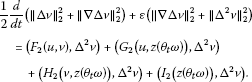(4.89)

By integrating by parts and employing Gagliardo-Nirenberg inequality, we have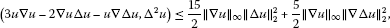(4.90)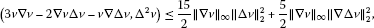(4.91)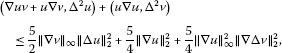(4.92)(4.93)

and(4.94)

Associating with (4.90)-(4.94), we get(4.95)

where ${c}_{11}=15c/8+5{c}^{2}/8$, ${c}_{12}=5/4$, ${c}_{13}=15c/2$, ${c}_{14}={c}^{2}/8$. As the considerations of above statements, we estimate the terms with random parameter in (4.88) and (4.89). They are bounded by(4.96)(4.97)

and(4.98)

Associating with (4.88)-(4.89), (4.95)-(4.98), we get

$\begin{array}{rl}\frac{d}{dt}{N}_{3}\left(t,\omega \right)\le & {c}_{11}{N}_{2}\left(t,\omega \right){N}_{3}\left(t,\omega \right)+{c}_{12}{N}_{2}\left(t,\omega \right)+{c}_{13}{N}_{3}\left(t,\omega \right)\\ +{c}_{14}{N}_{3}{\left(t,\omega \right)}^{2}+{c}_{15}{|y\left({\theta }_{t}\omega \right)|}^{2}{N}_{1}\left(t,\omega \right)\\ +{c}_{16}{|y\left({\theta }_{t}\omega \right)|}^{2}{N}_{3}\left(t,\omega \right)+{c}_{17}|y\left({\theta }_{t}\omega \right)|{N}_{3}\left(t,\omega \right)+{c}_{18}{|y\left({\theta }_{t}\omega \right)|}^{2},\end{array}$
(4.99)

where ${c}_{15}=915{c}^{2}/2\epsilon {\parallel h\parallel }_{{H}^{2}\left(\left[0,T\right]\right)}^{2}$, ${c}_{16}=max\left\{295/2\epsilon ,60\right\}{c}^{2}{\parallel h\parallel }_{{H}^{2}\left(\left[0,T\right]\right)}^{2}$, ${c}_{17}=\sqrt{5}c{\parallel h\parallel }_{{H}^{2}\left(\left[0,T\right]\right)}$, ${c}_{18}=\left(16+13\epsilon +13{\alpha }^{2}/\epsilon \right){\parallel h\parallel }_{{H}^{2}\left(\left[0,T\right]\right)}^{2}$. By Gronwall lemma from s to $t+1$, where $s\in \left[t,t+1\right]$ and $t\ge {T}_{2}\left(\omega \right)$ we have that

$\begin{array}{rl}{N}_{3}\left(t+1,\omega \right)\le & {e}^{{J}_{4}\left(\omega ,s,t+1\right)}{N}_{3}\left(s,\omega \right)+{c}_{12}{\int }_{s}^{t+1}{N}_{2}\left(\tau ,\omega \right){e}^{{J}_{4}\left(\omega ,\tau ,t+1\right)}\phantom{\rule{0.2em}{0ex}}d\tau \\ +{\int }_{s}^{t+1}\left({c}_{15}{|y\left({\theta }_{\tau }\omega \right)|}^{2}{N}_{1}\left(\tau ,\omega \right)+{c}_{18}{|y\left({\theta }_{\tau }\omega \right)|}^{2}\right){e}^{{J}_{4}\left(\omega ,\tau ,t+1\right)}\phantom{\rule{0.2em}{0ex}}d\tau ,\end{array}$
(4.100)

where(4.101)

Replacing ω by ${\theta }_{-t-1}\omega$ in (4.100), we have

$\begin{array}{r}{N}_{3}\left(t+1,{\theta }_{-t-1}\omega \right)\\ \phantom{\rule{1em}{0ex}}\le {e}^{{J}_{4}\left({\theta }_{-t-1}\omega ,s,t+1\right)}{N}_{3}\left(s,{\theta }_{-t-1}\omega \right)+{c}_{12}{\int }_{t}^{t+1}{N}_{2}\left(\tau ,{\theta }_{-t-1}\omega \right){e}^{{J}_{4}\left({\theta }_{-t-1}\omega ,\tau ,t+1\right)}\phantom{\rule{0.2em}{0ex}}d\tau \\ \phantom{\rule{2em}{0ex}}+{\int }_{t}^{t+1}\left({c}_{15}{|y\left({\theta }_{\tau -t-1}\omega \right)|}^{2}{N}_{1}\left(\tau ,{\theta }_{-t-1}\omega \right)+{c}_{18}{|y\left({\theta }_{\tau -t-1}\omega \right)|}^{2}\right){e}^{{J}_{4}\left({\theta }_{-t-1}\omega ,\tau ,t+1\right)}\phantom{\rule{0.2em}{0ex}}d\tau .\end{array}$
(4.102)

As the previous estimates, for $s\in \left[t,t+1\right]$, we get

$\begin{array}{rcl}{J}_{4}\left({\theta }_{-t-1}\omega ,s,t+1\right)& =& {\int }_{s}^{t+1}\left({c}_{11}{N}_{2}\left(\tau ,{\theta }_{-t-1}\omega \right)+{c}_{13}+{c}_{14}{N}_{3}\left(\tau ,{\theta }_{-t-1}\omega \right)\\ +{c}_{16}{|y\left({\theta }_{\tau -t-1}\omega \right)|}^{2}+{c}_{17}|y\left({\theta }_{\tau -t-1}\omega \right)|\right)\phantom{\rule{0.2em}{0ex}}d\tau \\ \le & {c}_{11}{\int }_{t}^{t+1}{N}_{2}\left(\tau ,{\theta }_{-t-1}\omega \right)\phantom{\rule{0.2em}{0ex}}d\tau +{c}_{13}+{c}_{14}{\int }_{t}^{t+1}{N}_{3}\left(\tau ,{\theta }_{-t-1}\omega \right)\phantom{\rule{0.2em}{0ex}}d\tau \\ +{c}_{16}{\int }_{t}^{t+1}{|y\left({\theta }_{\tau -t-1}\omega \right)|}^{2}\phantom{\rule{0.2em}{0ex}}d\tau +{c}_{17}{\int }_{t}^{t+1}|y\left({\theta }_{\tau -t-1}\omega \right)|\phantom{\rule{0.2em}{0ex}}d\tau \\ \le & {c}_{11}{r}_{5}\left(\omega \right)+{c}_{13}+{c}_{14}{r}_{12}\left(\omega \right)+{c}_{16}{\int }_{-1}^{0}{|y\left({\theta }_{\tau }\omega \right)|}^{2}\phantom{\rule{0.2em}{0ex}}d\tau +{c}_{17}{\int }_{-1}^{0}|y\left({\theta }_{\tau }\omega \right)|\phantom{\rule{0.2em}{0ex}}d\tau \\ \le & {c}_{11}{r}_{5}\left(\omega \right)+{c}_{13}+{c}_{14}{r}_{12}\left(\omega \right)+\frac{2{c}_{16}}{\alpha }{e}^{\frac{\alpha }{2}}{r}_{4}\left(\omega \right)+\frac{2{c}_{17}}{\alpha }{e}^{\frac{\alpha }{2}}{r}_{4}\left(\omega \right).\end{array}$
(4.103)

We denote

${r}_{13}\left(\omega \right)={c}_{11}{r}_{5}\left(\omega \right)+{c}_{13}+{c}_{14}{r}_{12}\left(\omega \right)+\frac{2{c}_{16}}{\alpha }{e}^{\frac{\alpha }{2}}{r}_{4}\left(\omega \right)+\frac{2{c}_{17}}{\alpha }{e}^{\frac{\alpha }{2}}{r}_{4}\left(\omega \right).$
(4.104)

Integrating (4.102) with respect to s on $\left[t,t+1\right]$ where $t\ge {T}_{2}\left(\omega \right)$, we get

$\begin{array}{rcl}{N}_{3}\left(t+1,{\theta }_{-t-1}\omega \right)& \le & {e}^{{r}_{13}\left(\omega \right)}{\int }_{t}^{t+1}{N}_{3}\left(s,{\theta }_{-t-1}\omega \right)\phantom{\rule{0.2em}{0ex}}ds+{c}_{12}{e}^{{r}_{13}\left(\omega \right)}{r}_{5}\left(\omega \right)\\ +{c}_{15}{e}^{{r}_{13}\left(\omega \right)}{r}_{3}\left(\omega \right){\int }_{-1}^{0}{|y\left({\theta }_{\tau }\omega \right)|}^{2}\phantom{\rule{0.2em}{0ex}}d\tau +{c}_{18}{e}^{{r}_{13}\left(\omega \right)}{\int }_{-1}^{0}{|y\left({\theta }_{\tau }\omega \right)|}^{2}\phantom{\rule{0.2em}{0ex}}d\tau \\ \le & {e}^{{r}_{13}\left(\omega \right)}{r}_{12}\left(\omega \right)+{c}_{12}{e}^{{r}_{13}\left(\omega \right)}{r}_{5}\left(\omega \right)+\frac{2{c}_{15}}{\alpha }{e}^{{r}_{13}\left(\omega \right)+\frac{\alpha }{2}}{r}_{3}\left(\omega \right){r}_{4}\left(\omega \right)\\ +\frac{2{c}_{18}}{\alpha }{e}^{{r}_{13}\left(\omega \right)+\frac{\alpha }{2}}{r}_{4}\left(\omega \right).\end{array}$
(4.105)

We denote

$\begin{array}{rcl}{r}_{14}\left(\omega \right)& =& {e}^{{r}_{13}\left(\omega \right)}{r}_{12}\left(\omega \right)+{c}_{12}{e}^{{r}_{13}\left(\omega \right)}{r}_{5}\left(\omega \right)+\frac{2{c}_{15}}{\alpha }{e}^{{r}_{13}\left(\omega \right)+\frac{\alpha }{2}}{r}_{3}\left(\omega \right){r}_{4}\left(\omega \right)\\ +\frac{2{c}_{18}}{\alpha }{e}^{{r}_{13}\left(\omega \right)+\frac{\alpha }{2}}{r}_{4}\left(\omega \right),\end{array}$
(4.106)

which implies that

${\parallel \mathrm{\nabla }\mathrm{\Delta }u\left(t,{\theta }_{-t}\omega ,\mathrm{\nabla }\mathrm{\Delta }{u}_{0}\left({\theta }_{-t}\omega \right)\right)\parallel }_{2}^{2}+{\parallel \mathrm{\nabla }\mathrm{\Delta }v\left(t,{\theta }_{-t}\omega ,\mathrm{\nabla }\mathrm{\Delta }{v}_{0}\left({\theta }_{-t}\omega \right)\right)\parallel }_{2}^{2}\le {r}_{14}\left(\omega \right),$
(4.107)

which completes the proof. □

## 5 Random attractors

In this section, we prove the existence of a $\mathcal{D}$-random attractor for the random dynamical system Ψ associated with the stochastic viscous coupled Camassa-Holm equation (3.1)-(3.5) with periodic boundary condition. It follows from Lemma 4.4 that Φ has a closed random absorbing set in $\mathcal{D}$, which along with the $\mathcal{D}$-pullback asymptotic compactness will imply the existence of a unique $\mathcal{D}$-random attractor. The $\mathcal{D}$-pullback asymptotic compactness of Φ is given below and will be proved by the compactness of embedding of Sobolev space.

Lemma 5.1 The random dynamical system Φ is $\mathcal{D}$-pullback asymptotically compact in ${H}^{2}\left(\left[0,T\right]\right)×{H}^{2}\left(\left[0,T\right]\right)$: that is, for P-a.e. $\omega \in \mathrm{\Omega }$, the sequence $\mathrm{\Phi }\left({t}_{n},{\theta }_{-{t}_{n}}\omega ,{\left({u}_{0,n}\left({\theta }_{-{t}_{n}}\omega \right),{v}_{0,n}\left({\theta }_{-{t}_{n}}\omega \right)\right)}^{T}\right)$ has a convergent subsequence in ${H}^{2}\left(\left[0,T\right]\right)×{H}^{2}\left(\left[0,T\right]\right)$ provided ${t}_{n}\to \mathrm{\infty }$, $B={\left\{B\left(\omega \right)\right\}}_{\omega \in \mathrm{\Omega }}\in \mathcal{D}$ and ${\left({u}_{0,n}\left({\theta }_{-{t}_{n}}\omega \right),{v}_{0,n}\left({\theta }_{-{t}_{n}}\omega \right)\right)}^{T}\in B\left({\theta }_{-{t}_{n}}\omega \right)$.

Proof Let ${t}_{n}\to \mathrm{\infty }$, $B={\left\{B\left(\omega \right)\right\}}_{\omega \in \mathrm{\Omega }}\in \mathcal{D}$ and ${\left({u}_{0,n}\left({\theta }_{-{t}_{n}}\omega \right),{v}_{0,n}\left({\theta }_{-{t}_{n}}\omega \right)\right)}^{T}\in B\left({\theta }_{-{t}_{n}}\omega \right)$. Then by Lemma 4.4, for P-a.e. $\omega \in \mathrm{\Omega }$, we have that

${\left\{\mathrm{\Phi }\left({t}_{n},{\theta }_{-{t}_{n}}\omega ,{\left({u}_{0,n}\left({\theta }_{-{t}_{n}}\omega \right),{v}_{0,n}\left({\theta }_{-{t}_{n}}\omega \right)\right)}^{T}\right)\right\}}_{n=1}^{\mathrm{\infty }}$

is bounded in ${H}^{2}\left(\left[0,T\right]\right)×{H}^{2}\left(\left[0,T\right]\right)$. Hence, there is ${\left(\xi ,\eta \right)}^{T}\in {H}^{2}\left(\left[0,T\right]\right)×{H}^{2}\left(\left[0,T\right]\right)$ such that, up to a subsequence,

$\mathrm{\Phi }\left({t}_{n},{\theta }_{-{t}_{n}}\omega ,{\left({u}_{0,n}\left({\theta }_{-{t}_{n}}\omega \right),{v}_{0,n}\left({\theta }_{-{t}_{n}}\omega \right)\right)}^{T}\right)\to {\left(\xi ,\eta \right)}^{T}$

weakly in ${H}^{2}\left(\left[0,T\right]\right)×{H}^{2}\left(\left[0,T\right]\right)$. On the other hand, by Lemma 4.1, Lemma 4.3 and Lemma 4.6, there exists a random variable $r\left(\omega \right)$ and $T=T\left(B,w\right)$ such that for all $t\ge T$,

${\parallel \mathrm{\Phi }\left(t,{\theta }_{-t}\omega ,{\left({u}_{0}\left({\theta }_{-t}\omega \right),{v}_{0}\left({\theta }_{-t}\omega \right)\right)}^{T}\right)\parallel }_{{H}^{3}\left(\left[0,T\right]\right)}^{2}\le r\left(\omega \right).$
(5.1)

Let $N=N\left(B,\omega \right)$ be large enough such that ${t}_{n}\ge T$ for $n\ge N$. Then by (5.1) we find that, for all $n\ge N$,

${\parallel \mathrm{\Phi }\left({t}_{n},{\theta }_{-{t}_{n}}\omega ,{\left({u}_{0,n}\left({\theta }_{-{t}_{n}}\omega \right),{v}_{0,n}\left({\theta }_{-{t}_{n}}\omega \right)\right)}^{T}\right)\parallel }_{{H}^{3}\left(\left[0,T\right]\right)}^{2}\le r\left(\omega \right).$
(5.2)

By the compactness of embedding ${H}^{3}\left(\left[0,T\right]\right)×{H}^{3}\left(\left[0,T\right]\right)↪↪{H}^{2}\left(\left[0,T\right]\right)×{H}^{2}\left(\left[0,T\right]\right)$, it follows from (5.2) that, up to a subsequence,

$\mathrm{\Phi }\left({t}_{n},{\theta }_{-{t}_{n}}\omega ,{\left({u}_{0,n}\left({\theta }_{-{t}_{n}}\omega \right),{v}_{0,n}\left({\theta }_{-{t}_{n}}\omega \right)\right)}^{T}\right)\to {\left(\xi ,\eta \right)}^{T}$

strongly in ${H}^{2}\left(\left[0,T\right]\right)×{H}^{2}\left(\left[0,T\right]\right)$ as desired. □

We are now in a position to present our main result: the existence of a $\mathcal{D}$-random attractor for Ψ in ${H}^{2}\left(\left[0,T\right]\right)×{H}^{2}\left(\left[0,T\right]\right)$.

Theorem 5.1 The random dynamical system Ψ has a unique $\mathcal{D}$-random attractor in ${H}^{2}\left(\left[0,T\right]\right)×{H}^{2}\left(\left[0,T\right]\right)$.

Proof Notice that Φ has a closed random absorbing set ${\left\{K\left(\omega \right)\right\}}_{\omega \in \mathrm{\Omega }}$ in $\mathcal{D}$ by Lemma 4.4, and is $\mathcal{D}$-pullback asymptotically compact in ${H}^{2}\left(\left[0,T\right]\right)×{H}^{2}\left(\left[0,T\right]\right)$ by Lemma 5.1. Hence, the existence of a unique $\mathcal{D}$-random attractor for Φ follows from Theorem 2.1 immediately. Then (3.19) implies that Ψ has a $\mathcal{D}$-random attractor in ${H}^{2}\left(\left[0,T\right]\right)×{H}^{2}\left(\left[0,T\right]\right)$. □

We have checked the persistence of global attractor of viscous coupled Camassa-Holm equation with periodic boundary condition under a white noise.

## References

1. Camassa R, Holm DD: An integrable shallow water equation with peaked solutions. Phys. Rev. Lett. 1993, 71: 1661–1664. 10.1103/PhysRevLett.71.1661

2. Camassa R, Holm DD, Hyman JM: A new integrable shallow water equation. Adv. Appl. Mech. 1994, 31: 1–33.

3. Dullin HR, Gottwald GA, Holm DD: On asymptotically equivalent shallow water wave equations. Phys. D 2004, 190: 1–14. 10.1016/j.physd.2003.11.004

4. Dullin HR, Gottwald GA, Holm DD: CH, Korteweg-de Vries-J-5 and other asymptotically equivalent equations for shallow water waves. Fluid Dyn. Res. 2003, 33: 73–95. 10.1016/S0169-5983(03)00046-7

5. Constantin A, Strauss W: A stability of the Camassa-Holm solitons. J. Nonlinear Sci. 2002, 12: 415–422. 10.1007/s00332-002-0517-x

6. Bressan A, Constantin A: Global conservative solutions of the Camassa-Holm equation. Arch. Ration. Mech. Anal. 2007, 183: 215–239. 10.1007/s00205-006-0010-z

7. Constantin A, Strauss W: Stability of peakons. Commun. Pure Appl. Math. 2000, 53: 603–610. 10.1002/(SICI)1097-0312(200005)53:5<603::AID-CPA3>3.0.CO;2-L

8. Constantin A, Escher J: Global existence and blow-up for a shallow water equation. Ann. Sc. Norm. Super. Pisa, Cl. Sci. 1998, 26: 303–328.

9. Rodriguez-Blanco G: On the cauchy problem for the Camassa-Holm equation. Nonlinear Anal. 2001, 46: 309–327. 10.1016/S0362-546X(01)00791-X

10. Constantin A, Escher J: Wave breaking for nonlinear nonlocal shallow water equations. Acta Math. 1998, 181: 229–243. 10.1007/BF02392586

11. Xin ZP, Zhang P: On the weak solutions to a shallow water equation. Commun. Pure Appl. Math. 2000, 53: 1411–1433. 10.1002/1097-0312(200011)53:11<1411::AID-CPA4>3.0.CO;2-5

12. Bressan A, Constantin A: Global dissipative solutions of the Camassa-Holm equation. Anal. Appl. 2007, 5: 1–27. 10.1142/S0219530507000857

13. Fu Y, Qu C: Well posedness and blow-up solution for a new coupled Cammassa-Holm equations with peakons. J. Math. Phys. 2009., 50: Article ID 012906

14. Tian L, Xu Y: Attractor for a viscous coupled Camassa-Holm equation. Adv. Differ. Equ. 2010., 2010: Article ID 512812

15. Tian L, Xu Y, Zhou J: Attractor for the viscous two-component Camassa-Holm equation. Nonlinear Anal. 2012, 13: 1115–1129. 10.1016/j.nonrwa.2011.09.005

16. Arnold L: Random Dynamical Systems. Springer, Berlin; 1998.

17. Crauel H, Flandoli F: Attractors for random dynamical systems. Probab. Theory Relat. Fields 1994, 100: 365–393. 10.1007/BF01193705

18. Chueshov I: Monotone Random Systems Theory and Applications. Springer, Berlin; 2002.

19. Da Prato G, Zabczyk J: Stochastic Equations in Infinite Dimensions. Cambridge University Press, Cambridge; 1992.

20. Øksendal B: Stochastic Differential Equations. Springer, Berlin; 2000.

21. Crauel H, Debussche A, Flandoli F: Random attractor. J. Dyn. Differ. Equ. 1997, 9: 307–341. 10.1007/BF02219225

22. Flandoli F, Schmalfuß B: Random attractors for the 3D stochastic Navier-Stokes equation with multiplicative noise. Stoch. Stoch. Rep. 1996, 59: 21–45.

23. Babin AV, Vishik MI: Attractors of Evolution Equations. North-Holland, Amsterdam; 1992.

24. Hale JK: Asymptotic Behaviour of Dissipative Systems. Am. Math. Soc., Providence; 1988.

25. Robinson JC: Infinite-Dimensional Dynamical Systems. Cambridge University Press, Cambridge; 2001.

26. Sell R, You Y: Dynamics of Evolutionary Equations. Springer, New York; 2002.

27. Teman R: Infinite-Dimensional Dynamical Systems in Mechanics and Physics. Springer, New York; 1998.

28. Bates PW, Lisei H, Lu K: Attractors for stochastic lattice dynamical systems. Stoch. Dyn. 2006, 6: 1–21. 10.1142/S0219493706001621

29. Bates PW, Lu K, Wang B: Random attractors for stochastic reaction-diffusion equations on unbounded domains. J. Differ. Equ. 2009, 246: 845–869. 10.1016/j.jde.2008.05.017

30. Caraballo T, Langa JA, Robinson JC: A stochastic pitchfork bifurcation in a reaction-diffusion equation. Proc. R. Soc. Lond. Ser. A 2001, 457: 2041–2061. 10.1098/rspa.2001.0819

31. Han X, Shen W, Zhou S: Random attractors for stochastic lattice dynamical systems in weighted spaces. J. Differ. Equ. 2011, 250: 1235–1266. 10.1016/j.jde.2010.10.018

32. Wang B: Random attractors for the stochastic Benjamin-Bona-Mahony equation on unbounded domians. J. Differ. Equ. 2009, 246: 2506–2537. 10.1016/j.jde.2008.10.012

33. Wang Z, Zhou S, Gu A: Random attractor of the stochastic strongly damped wave equation. Commun. Nonlinear Sci. Numer. Simul. 2012, 17: 1649–1658. 10.1016/j.cnsns.2011.09.001

34. Wang Z, Zhou S, Gu A: Random attractor for a stochastic damped wave equation with multiplicative noise on unbounded domains. Nonlinear Anal. 2011, 12: 3468–3482. 10.1016/j.nonrwa.2011.06.008

35. Wang X, Li S, Xu D: Random attractors for second-order stochastic lattice dynamical systems. Nonlinear Anal. 2010, 72: 483–494. 10.1016/j.na.2009.06.094

36. Crauel H: Random point attractors versus random set attractors. J. Lond. Math. Soc. 2002, 63: 413–427.

## Acknowledgements

The authors would like to thank reviewers for the valuable suggestions and comments. The paper is supported by the National Natural Science Foundation (No. 11171115).

## Author information

Authors

### Corresponding author

Correspondence to Zhengrong Liu.

### Competing interests

The authors declare that they have no competing interests.

### Authors’ contributions

ZH mainly completed the proofs appearing in the manuscript and drafted the manuscript. HT participated in completeness of the proofs. ZL provided some important intellectual contents and revised the manuscript critically. All authors read and approved the final manuscript.

## Rights and permissions

Reprints and Permissions

Huang, Z., Tang, H. & Liu, Z. Random attractor for a stochastic viscous coupled Camassa-Holm equation. J Inequal Appl 2013, 201 (2013). https://doi.org/10.1186/1029-242X-2013-201